## Propositional-Logic

 Question 1

Consider the first order predicate formula φ:

∀x[(∀z z|x ⇒ ((z = x) ∨ (z = 1))) ⇒ ∃w (w > x) ∧ (∀z z|w ⇒ ((w = z) ∨ (z = 1)))]

Here 'a|b' denotes that 'a divides b', where a and b are integers. Consider the following sets:

S1.  {1, 2, 3, ..., 100}
S2.  Set of all positive integers
S3. Set of all integers

Which of the above sets satisfy φ?

 A S1 and S3 B S1, S2 and S3 C S2 and S3 D S1 and S2
Engineering-Mathematics       Propositional-Logic       GATE 2019
Question 1 Explanation:
The first order logic gives the meaning that if z is a prime number then there exists another prime number in the set which is larger than it.
One of the case:
If -7 is a number which is prime (either divided by -7 or 1 only). then there exists some number like -3 which is larger than -7 also satisfy the property (either divided by -3 or 1 only).
So, S3 is correct
It's true for all integers too.
 Question 2

Consider the first-order logic sentence

φ ≡ ∃s∃t∃u∀v∀w∀x∀y ψ(s,t,u,v,w,x,y)

where ψ(s,t,u,v,w,x,y) is a quantifier-free first-order logic formula using only predicate symbols, and possibly equality, but no function symbols. Suppose φ has a model with a universe containing 7 elements.

Which one of the following statements is necessarily true?

 A There exists at least one model of φ with universe of size less than or equal to 3. B There exists no model of φ with universe of size less than or equal to 3. C There exists no model of φ with universe of size greater than 7. D Every model of φ has a universe of size equal to 7.
Engineering-Mathematics       Propositional-Logic       GATE 2018
Question 2 Explanation:
φ = ∃s∃t∃u∀v∀w∀x∀y ψ (s,t,u,v,w,x,y)
"∃" there exists quantifier decides whether a sentence belong to the model or not.
i.e., ~∃ will make it not belong to the model. (1) We have ‘7’ elements in the universe, So max. size of universe in a model = ‘7’
(2) There are three '∃' quantifiers, which makes that a model have atleast “3” elements. So, min. size of universe in model = ‘7’.
(A) is False because: (2)
(B) is true
(C) is false because of (1)
(D) is false, because these all models with size {3 to 7} not only ‘7’.
 Question 3
The statement (¬p) ⇒ (¬q) is logically equivalent to which of the statements below?
I. p ⇒ q
II. q ⇒ p
III. (¬q) ∨ p
IV. (¬p) ∨ q
 A I only B I and IV only C II only D II and III only
Engineering-Mathematics       Propositional-Logic       GATE 2017 [Set-1]       Video-Explanation
Question 3 Explanation:
Method 1:
Construct Truth tables:
~p ⇒ ~q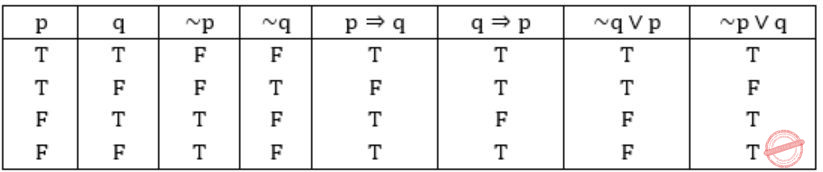II, III are equivalent to (~p) ⇒ (~q)
Method 2:
(I) p⇒q ≡ ~p∨q
(II) q⇒p ≡ ~q∨p
(III) (~q) ∨ p ≡ ~q∨p
(IV) (~p) ∨ p ≡ ~p∨q
Also, from question:
(~p) ⇒ (~q)
≡ p∨~q
So, (II) & (III) are equivalent to the statement given in question.
 Question 4
Consider the following expressions:
(i) false
(ii) Q
(iii) true
(iv) P ∨ Q
(v) ¬Q ∨ P
The number of expressions given above that are logically implied by P ∧ (P ⇒ Q) is _________.
 A 4 B 5 C 6 D 7
Engineering-Mathematics       Propositional-Logic       GATE 2016 [Set-2]       Video-Explanation
Question 4 Explanation:
The expression is logically implied by P ∧ (P → Q) means
(P ∧ (P → Q))→ expression is a tautology. So we have to find
How many tautological formulas are there for the given inputs.
(P ∧ (P → Q)) → True is always tautology
(P ∧ (P → Q)) → False is not a tautology
(P ∧ (P → Q)) → Q is a tautology
(P ∧ (P → Q)) → ¬Q ∨ P is a tautology
(P ∧ (P → Q)) → P ∨ Q is a tautology
So there are 4 expressions logically implied by (P ∧ (P → Q))
 Question 5

Which one of the following well-formed formulae in predicate calculus is NOT valid?

 A (∀x p(x) ⇒ ∀x q(x)) ⇒ (∃x ¬p(x) ∨ ∀x q(x)) B (∃x p(x) ∨ ∃x q(x)) ⇒ ∃x (p(x) ∨ q(x)) C ∃x (p(x) ∧ q(x)) ⇒ (∃x p(x) ∧ ∃x q(x)) D ∀x (p(x) ∨ q(x)) ⇒ (∀x p(x) ∨ ∀x q(x))
Engineering-Mathematics       Propositional-Logic       GATE 2016 [Set-2]       Video-Explanation
Question 5 Explanation:
For the formulae to be valid there should not be implication like T → F.
But in option (D), we can generate T → F.
Hence, not valid.
 Question 6

Which one of the following well formed formulae is a tautology?

 A ∀x ∃y R(x,y) ↔ ∃y ∀x R(x,y) B (∀x [∃y R(x,y) → S(x,y)]) → ∀x∃y S(x,y) C [∀x ∃y (P(x,y) → R(x,y)] ↔ [∀x ∃y (¬ P(x,y)∨R(x,y)] D ∀x ∀y P(x,y) → ∀x ∀y P(y,x)
Engineering-Mathematics       Propositional-Logic       GATE 2015 [Set-2]
Question 6 Explanation:
Since P→R = ¬P∨R
[∀x ∃y (P(x,y) → R(x,y)] ↔ [∀x ∃y (¬ P(x,y)∨R(x,y)] is a tautology.
 Question 7

Consider the following logical inferences.

I1: If it rains then the cricket match will not be played.
The cricket match was played.
Inference: There was no rain.
I2: If it rains then the cricket match will not be played.
It did not rain.
Inference: The cricket match was played.

Which of the following is TRUE?

 A Both I1 and I2 are correct inferences B I1 is correct but I2 is not a correct inference C I1 is not correct but I2 is a correct inference D Both I1 and I2 are not correct inferences
Engineering-Mathematics       Propositional-Logic       GATE 2012
Question 7 Explanation:
I1: If it rains then the cricket match will not be played.
The cricket match was played.
Let p = it rains
q = playing cricket/ match played
If (it rains) then (the match will not be played)
p ⇒ (∼q)
Inference: There was no rain. (i.e., p = F)
So for any F ⇒ (∼q) is true.
So this inference is valid.
I2: If it rains then the cricket match will not be played.
It did not rain.
p ⇒ (∼q)
Inference: The cricket match was played.
q = T
p ⇒ (∼q)
p ⇒ (∼T)
p ⇒ F
This is false for p = T, so this is not true.
 Question 8

What is the correct translation of the following statement into mathematical logic?

“Some real numbers are rational”

 A ∃x (real(x) ∨ rational(x)) B ∀x (real(x) → rational(x)) C ∃x (real(x) ∧ rational(x)) D ∃x (rational(x) → real(x))
Engineering-Mathematics       Propositional-Logic       GATE 2012
Question 8 Explanation: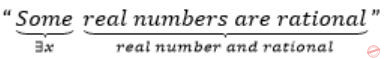∃x (real(x) ∧ rational(x))
(A) ∃x(real(x) ∨ rational(x))
means There exists some number, which are either real or rational.
(B) ∀x (real(x)→rational(x))
If a number is real then it is rational.
(D) ∃x (rational(x)→real(x))
There exists a number such that if it is rational then it is real.
 Question 9

Which one of the following options is CORRECT given three positive integers x,y and z, and a predicate

P(x) = ¬(x=1)∧∀y(∃z(x=y*z) ⇒ (y=x)∨(y=1))
 A P(x) being true means that x is a prime number B P(x) being true means that x is a number other than 1 C P(x) is always true irrespective of the value of x D P(x) being true means that x has exactly two factors other than 1 and x
Engineering-Mathematics       Propositional-Logic       GATE 2011
Question 9 Explanation:
Statement: x is not equal to 1 and if there exists some z for all y such that product of y and z is x, then y is either the number itself or 1.
This is the definition of prime numbers.
 Question 10

Suppose the predicate F(x, y, t) is used to represent the statement that person x can fool person y at time t. Which one of the statements below expresses best the meaning of the formula ∀x∃y∃t(¬F(x,y,t))?

 A Everyone can fool some person at some time B No one can fool everyone all the time C Everyone cannot fool some person all the time D No one can fool some person at some time
Engineering-Mathematics       Propositional-Logic       GATE 2010
Question 10 Explanation:
F(x,y,t) ⇒ Person 'x' can fool person 'y' at time 't'.
For better understanding propagate negation sign outward by applying Demorgan's law.
∀x∃y∃t(¬F(x, y, t)) ≡ ¬∃x∀y∀t(F(x,y,t))
Now converting ¬∃x∀y∀t(F(x,y,t)) to English is simple.
¬∃x∀y∀t(F(x,y,t)) ⇒ There does not exist a person who can fool everyone all the time.
Which means "No one can fool everyone all the time".
Hence, Option (B) is correct.
 Question 11

Which one of the following is the most appropriate logical formula to represent the statement?

`      “Gold and silver ornaments are precious”.`

The following notations are used:

```
G(x): x is a gold ornament
S(x): x is a silver ornament
P(x): x is precious```
 A ∀x(P(x) → (G(x) ∧ S(x))) B ∀x((G(x) ∧ S(x)) → P(x)) C ∃x((G(x) ∧ S(x)) → P(x) D ∀x((G(x) ∨ S(x)) → P(x))
Engineering-Mathematics       Propositional-Logic       GATE 2009
Question 11 Explanation:
Interpreting the options will lead to
(A) for all ornaments, if it is precious then they should be gold and silver.
But, given statement does not says that, “ only gold and silver are precious “ . So this is wrong.
(B) For all ornaments, which contains gold and silver are precious.Which is only the shaded region in the venn diagrams. But, it misses p,r regions. So, this is wrong option.
C) Some ornaments, which are gold and silver are precious. It is false, because all gold or silver ornaments are precious.
D) For all ornaments, Any ornament which is gold or silver is precious. Which is true.
 Question 12

The binary operation □ is defined as follows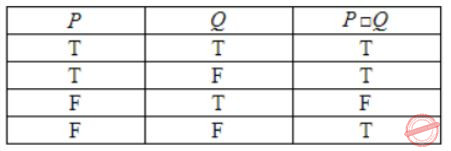Which one of the following is equivalent to P ∨ Q ?

 A ¬Q□¬P B P□¬Q C ¬P□Q D ¬P□¬Q
Engineering-Mathematics       Propositional-Logic       GATE 2009
Question 12 Explanation:
The options are simple to draw the truth table then go with the corresponding options.P∨Q = P□️Q
So, option B is correct.
 Question 13

Consider the following well-formed formulae:

I. ¬∀x(P(x))
II. ¬∃(P(x))
III. ¬∃(¬P(x))
IV. ∃x(¬(P(x))

Which of the above are equivalent?

 A I and III B I and IV C II and III D II and IV
Engineering-Mathematics       Propositional-Logic       GATE 2009
Question 13 Explanation:
I) ¬∀x(P(x)) = ∃x(¬P(x)) [Demorgan's Law]
II ) ¬∃x(P(x))= ∀x(~P(x))
III) ¬∃x(¬P(x)) = ∀x(P(x))
 Question 14

Let fsa and pda be two predicates such that fsa(x) means x is a finite state automaton, and pda(y) means that y is a pushdown automaton. Let equivalent be another predicate such that equivalent (a, b) means a and b are equivalent. Which of the following first order logic statements represents the following:

Each finite state automaton has an equivalent pushdown automaton

 A (∀x fsa(x)) ⇒ (∃y pda(y) ∧ equivalent(x,y)) B ∼∀y(∃x fsa(x) ⇒ pda(y) ∧ equivalent(x,y)) C ∀x ∃y(fsa(x) ∧ pda(y) ∧ equivalent(x,y)) D ∀x ∃y(fsa(y)∧ pda(x) ∧ equivalent(x,y))
Engineering-Mathematics       Propositional-Logic       GATE 2008
Question 14 Explanation:
Go through the options.
Option A:
If everything is a FSA. Then there exists an equivalent PDA for everything.
Option B:
Not for the case Y, if there exists a FSA then it can have equivalent PDA.
Option C:
Everything is a PDA and consists equivalent PDA.
Option D:
Everything is a PDA and has exist an equivalent FSA. In option A we are getting the equivalent of a and b.
So answer is option A.
 Question 15

P and Q are two propositions. Which of the following logical expressions are equivalent?

I. P∨∼Q
II. ∼(∼P∧Q)
III. (P∧Q)∨(P∧∼Q)∨(∼P∧∼Q)
IV. (P∧Q)∨(P∧∼Q)∨(∼P∧Q)
 A Only I and II B Only I, II and III C Only I, II and IV D All of I, II, III and IV
Engineering-Mathematics       Propositional-Logic       GATE 2008
Question 15 Explanation:
I. P∨∼Q (✔️)
II. ∼(∼P∧Q)⇒(P∨∼Q)≡I (✔️)
III. (P×Q)∨(P×∼Q)∨(∼P×∼Q)
P∧(Q∨∼Q)∨(∼P∧∼Q)
P∨(∼P×∼Q)
(P∨∼P)×(P∨∼Q)
(P∨∼Q)≡I=II (✔️)
IV. (P×Q)∨(P∧∼Q)∨(∼P×Q)
P×(Q∨∼Q)∨(∼P∧Q)
P∨(∼P×Q)
(P∨∼P)×(P∨Q)
(P∨Q)≠I (❌)
So I≡II≡III (✔️)
 Question 16

Let Graph(x) be a predicate which denotes that x is a graph. Let Connected(x) be a predicate which denotes that x is connected. Which of the following first order logic sentences DOES NOT represent the statement: “Not every graph is connected”?

 A ¬∀x (Graph (x) ⇒ Connected (x)) B ¬∃x (Graph (x) ∧ ¬Connected (x)) C ¬∀x (¬Graph (x) ∨ Connected (x)) D ∀x (Graph (x) ⇒ ¬Connected (x))
Engineering-Mathematics       Propositional-Logic       GATE 2007
Question 16 Explanation:
Option (A) and (C) are same, because in option (C) the given expression is
Given expression is
¬∀x(¬Graph(x) ∨ Connected(x)
which can be rewritten as,
¬∀x(Graph(x) ⇒ Connected(x)
which is equivalent to option (A)
(∵ ¬p∨q ≡ p→q)
So, option (A) and (C) cannot be the answer.
Coming to option (B), the given expression is,
∃x (Graph (x) ∧ ¬Connected (x))
"There exist some graph which is not connected", which is equivalent in saying that "Not every graph is connected".
Coming to option (D),
For all x graph is not connected, which is not correct.
Hence, option (D) is the answer.
 Question 17

Which one of the first order predicate calculus statements given below correctly express the following English statement?

`     Tigers and lions attack if they are hungry or threatened.`
 A ∀x [(tiger(x) ∧ lion(x)) → {(hungry(x) ∨ threatened(x)) → attacks(x)}] B ∀x [(tiger(x) ∨ lion(x)) → {(hungry(x) ∨ threatened(x)) ∧ attacks(x)}] C ∀x [(tiger(x) ∨ lion(x)) → {(attacks(x) → (hungry (x)) ∨ threatened (x))}] D ∀x [(tiger(x) ∨ lion(x)) → {(hungry(x) ∨ threatened(x)) → attacks(x)}]
Engineering-Mathematics       Propositional-Logic       GATE 2006
Question 17 Explanation:
Tigers and lions attack if they are hungry (or) threatened.
Here we have two cases.
i) If Tiger is hungry (or) threaten that will attack.
ii) If Lion is hungry (or) threaten that will attack.
If Tiger is hungry (or) threaten then both lion and tiger will not attack only Tiger will attack and vice-versa.
∀x[(tiger(x) ∨ lion(x)) → {(hungry(x) ∨ threatened(x)) → attacks(x)}]
Note: Don’t confuse with the statement Tiger and Lion.
 Question 18

Consider the following propositional statements:

P1 : ((A ∧ B) → C)) ≡ ((A → C) ∧ (B → C))
P2 : ((A ∨ B) → C)) ≡ ((A → C) ∨ (B → C))

Which one of the following is true?

 A P1 is a tautology, but not P2 B P2 is a tautology, but not P1 C P1 and P2 are both tautologies D Both P1 and P2 are not tautologies
Engineering-Mathematics       Propositional-Logic       GATE 2006
Question 18 Explanation:
It’s better to draw truth table such thatBoth P1 and P2 are not Tautologies.
 Question 19

Let P, Q and R be three atomic prepositional assertions. Let X denote (P ∨ Q) → R and Y denote (P → R) ∨ (Q → R). Which one of the following is a tautology?

 A X ≡ Y B X → Y C Y → X D ¬Y → X
Engineering-Mathematics       Propositional-Logic       GATE 2005
Question 19 Explanation:
X: (P∨Q) → R
⇒ ∼(P∨Q) ∨ R
⇒ (∼P∧∼Q) ∨ R
⇒ (∼P∨R) × (∼Q∨R)
⇒ (P→R) ∧ (Q→R)
Option B: X→Y
[(P→R) × (Q→R)] → [(P→R) ∨ (Q→R)]
∼[(P→R) × (Q→R) ∨ (P→R) ∨ (Q→R)]
[∼(P→R) ∨ ∼(Q→R)] ∨ [(P→R) ∨ (Q→R)]
[∼(P→R) ∨ (P→R)] ∨ [∼(P→R) ∨ (Q→R)] ∨ [(Q→R) ∨ (P→R)] ∨ [∼(Q→R) ∨ (Q→R)]
T ∨ [∼(P→R) ∨ (Q→R)] ∨ [(Q→R) ∨ (P→R)] V T
T (✔️)
 Question 20

What is the first order predicate calculus statement equivalent to the following?

`   Every teacher is liked by some student  `
 A ∀(x) [teacher(x) → ∃ (y) [student(y) → likes (y, x)]] B ∀(x) [teacher(x) → ∃ (y) [student(y) ∧ likes (y, x)]] C ∃(y) ∀(x) [teacher(x) → [student(y) ∧ likes (y, x)]] D ∀(x) [teacher(x) ∧ ∃ (y)[student(y) → likes (y, x)]]
Engineering-Mathematics       Propositional-Logic       GATE 2005
Question 20 Explanation:
Option A: If x is a teacher, then there exist a y such that if y is a student , then y likes x.
Option B: If x is a teacher, then there exists some y, who is a student and like x. (✔️)
Option C: There exists a student who likes all teachers.
Option D: If x is a teacher and then there exists some y, if y is a student then y likes x.
 Question 21

Identify the correct translation into logical notation of the following assertion.

`      "Some boys in the class are taller than all the girls"  `

Note: taller(x,y) is true if x is taller than y.

 A (∃x) (boy(x) → (∀y) (girl(y) ∧ taller(x,y))) B (∃x) (boy(x) ∧ (∀y) (girl(y) ∧ taller(x,y))) C (∃x) (boy(x) → (∀y) (girl(y) → taller(x,y))) D (∃x) (boy(x) ∧ (∀y) (girl(y) → taller(x,y)))
Engineering-Mathematics       Propositional-Logic       GATE 2004
Question 21 Explanation:
Don't confuse with '∧' and '→'
'∧' → predicts statements are always true, no matter the value of x.
'→' → predicts there is no need of left predicate to be true always, but whenever it becomes true, then right predicate must be true.
Option D:
There exists a some boys who are taller than of all girls y.
 Question 22

The following propositional statement is (P → (Q v R)) → ((P v Q) → R)

 A satisfiable but not valid B valid C a contradiction D None of the above
Engineering-Mathematics       Propositional-Logic       GATE 2004
Question 22 Explanation:
(P→(Q∨R)) → ((P∨Q)→R)
If P=T; Q=T; R=T
(P→(T∨T)) → ((T∨T)→R)
(P→T) → (T→R)
(T→T) → (T→T)
T→T
T(Satisfiable)
 Question 23

Which of the following is a valid first order formula? (Here α and β are first order formulae with x as their only free variable)

 A ((∀x)[α] ⇒ (∀x)[β]) ⇒ (∀x)[α⇒β] B (∀x)[α] ⇒ (∃x)[α ∧ β] C ((∀x)[α ∨ β] ⇒ (∃x)[α] ⇒ (∀x)[α] D (∀x)[α ⇒ β] ⇒ ((∀x)[α] ⇒ (∀x)[β])
Engineering-Mathematics       Propositional-Logic       GATE 2003
Question 23 Explanation:
Option D is valid.
Here, α, β are holding values of x. Then and RHS saying that α holding the value of x and β is holding value of x.
Then LHS ⇒ RHS.
 Question 24

Consider the following formula a and its two interpretations I1 and I2

```α: (∀x)[Px ⇔ (∀y)[Qxy ⇔ ¬Qyy]] ⇒ (∀x)[¬Px]
I1: Domain: the set of natural numbers
Px ≡ 'x is a prime number'
Qxy ≡ 'y divides x'
I2: same as I1 except that Px = 'x is a composite number'.```

Which of the following statements is true?

 A I1 satisfies α, I2 does not B I2 satisfies α, I1 does not C Neither I2 nor I1 satisfies α D Both I1 and I2 satisfy α
Engineering-Mathematics       Propositional-Logic       GATE 2003
Question 24 Explanation:
Given that:
(∀x)[Px ⇔ (∀y)[Qxy ⇔ ¬Qyy]] ⇒(∀x)[¬Px]
Qyy is always true, because y divide y, then ¬Qyy is false.
∀x[(P(x) ⇔ ∀y [Qxy ⇔ False]]
∀y [Qxy ⇔ False] can be written as ∀y[¬axy]
⇒(∀x)[P(x) ⇔ ∀y[¬Qxy]]
Here, ¬Qxy says that y doesnot divides x, which is not always be true.
For example, if x=y then it is false then ∀y[¬Qxy] is not true for all values of y.
⇒(∀x)[P(x) ⇔ False]
⇒(∀x)[¬P(x) = RHS]
LHS = RHS
⇒ Irrespective of x, whether x is prime of composite number I1 and I2 satisfies α.
 Question 25

The following resolution rule is used in logic programming.

`Derive clause (P ∨ Q) from clauses (P ∨ R), (Q ∨ ¬R) `

Which of the following statements related to this rule is FALSE?

 A ((P ∨ R) ∧ (Q ∨ ¬R)) ⇒ (P ∨ Q) is logically valid B (P ∨ Q) ⇒ ((P ∨ R) ∧ (Q ∨ ¬R)) is logically valid C (P ∨ Q) is satisfiable if and only if (P∨R) ∧ (Q∨¬R) is satisfiable D (P ∨ Q) ⇒ FALSE if and only if both P and Q are unsatisfiable
Engineering-Mathematics       Propositional-Logic       GATE 2003
Question 25 Explanation:
(P ∨ Q) ⇒ ((P ∨ R) ∧ (Q ∨ ¬R))It is may be True (or) False depending on values. So this is not valid.
 Question 26

“If X then Y unless Z” is represented by which of the following formulas in prepositional logic? (“¬“, is negation, “∧” is conjunction, and “→” is implication)

 A (X∧¬Z)→Y B (X∧Y)→¬Z C X→(Y∧¬Z) D (X→Y)∧¬Z
Engineering-Mathematics       Propositional-Logic       GATE 2002
Question 26 Explanation:
"If X then Y unless Z" ⇒ ¬Z → (X→Y)
⇒ Z ∨ ¬X ∨ Y
⇒ ¬X ∨ Z ∨ Y
Option A:
(X ∧ ¬Z) → Y = ¬(X ∧ ¬Z ) ∨ Y = ¬X ∨ Z ∨ Y Hence, option (A) is correct.
 Question 27

Consider two well-formed formulas in prepositional logic

`   F1: P ⇒ ¬P          F2: (P⇒¬P)∨(¬P⇒P)  `

Which of the following statements is correct?

 A F1 is satisfiable, F2 is valid B F1 unsatisfiable, F2 is satisfiable C F1 is unsatisfiable, F2 is valid D F1 and F2 are both satisfiable
Engineering-Mathematics       Propositional-Logic       GATE 2001
Question 27 Explanation:F1 is satisfiable; F2 is valid.
 Question 28

Let a, b, c, d be propositions. Assume that the equivalence a ↔ (b ∨ -b) and b ↔ c hold. Then the truth-value of the formula (a ∧ b) → (a ∧ c) ∨ d is always

 A True B False C Same as the truth-value of b D Same as the truth-value of d
Engineering-Mathematics       Propositional-Logic       GATE 2000
Question 28 Explanation:
a ↔ (b ∨-b) and b ↔ c
Given ⇒ (a∧b) → (a∧c) ∨d
⇒ (a∧b) → (a∧c) ∨d (b⇔c)
⇒ T∨d
⇒ T
 Question 29

(a) Show that the formula [(~p ∨ q) ⇒ (q ⇒ p)] is not a tautology.
(b) Let A be a tautology and B be any other formula. Prove that (A ∨ B) is a tautology.

 A Theory Explanation.
Engineering-Mathematics       Propositional-Logic       GATE 1999
 Question 30

What is the converse of the following assertion?

`  I stay only if you go. `
 A I stay if you go B If I stay then you go C If you do not go then I do not stay D If I do not stay then you go
Engineering-Mathematics       Propositional-Logic       GATE 1998
Question 30 Explanation:
"I stay only you go" = "If I stay then you go"
⇒ i.e., A→B
Where A = If I stay; B = you go
Converse for (A→B) is (B→A)
⇒ If you go then I stay.
 Question 31

Which of the following propositions is a tautology?

 A (p ∨ q) → p B p ∨ (q → p) C p ∨ (p → q) D p → (p → q)
Engineering-Mathematics       Propositional-Logic       GATE 1997
Question 31 Explanation: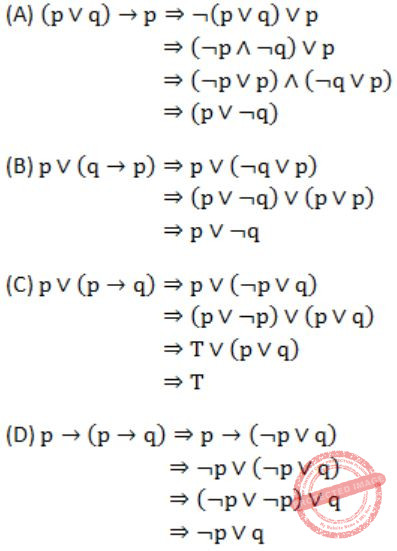Question 32

Which of the following is false? Read ∧ as AND, ∨ as OR, ~ as NOT, → as one way implication and ↔ as two way implication.

 A ((x → y) ∧ x) → y B ((x → y) ∧ (x ∧ y)) → x C (x → (x ∨ ψ)) D ((x ∨ y) ↔ (x → y)
Engineering-Mathematics       Propositional-Logic       GATE 1996
Question 32 Explanation:
When x = F and y = F
then option (D) will be False.
 Question 33

Obtain the principal (canonical) conjunctive normal form of the propositional formula

`  (p ∧ q) V (¬q ∧ r) `

Where ‘∧’ is logical and, ‘v’ is inclusive or and ¬ is negation.

 A Theory Explanation.
Engineering-Mathematics       Propositional-Logic       GATE 1995
 Question 34

(a) Let * be a Boolean operation defined asIf C = A * B then evaluate and fill in the blanks:
(i) A * A = _______
(ii) C * A = _______
(b) Solve the following boolean equations for the values of A, B and C:A Theory Explanation.
Engineering-Mathematics       Propositional-Logic       GATE 1994
 Question 35

Which one of the following predicate formulae is NOT logically valid?
Note that W is a predicate formula without any free occurrence of x.

 A ∃x(p(x) → W) ≡ ∀x p(x) → W B ∀x(p(x) → W) ≡ ∀x p(x) → W C ∃x(p(x) ∧ W) ≡ ∃x p(x) ∧ W D ∀x(p(x) ∨ W) ≡ ∀x p(x) ∨ W
Engineering-Mathematics       Propositional-Logic       GATE 2020
Question 35 Explanation:
Basic Rules:
~p→q ≡ ~p∨q
Demorgan laws:
~(∀x(a(x)) ≡ ∃x~a(x)
~(∃x(a(x)) ≡ ∀x~a(x)
(A) ∃x(p(x)→w) ≡ ∀x p(x)→w
LHS: ∃x(p(x)→w) ≡ ∃x(~p(x)∨w)
≡ ∃x(~p(x))∨w
Demorgan’s law:
~(∀x(a(x)) = ∃x ~ a(x)
≡ ~(∀x P(x)) ∨ w
≡ (∀x) P(x) → w ≡ RHS
It’s valid.
(B) ∀x(P(x) → w) ≡ ∀x(~P(x) ∨ w)
≡ ∀x(~P(x)) ∨ w
≡ ~(∃x P(x)) ∨ w
≡ ∃x P(x) → w
This is not equal to RHS.
(C) ∃x(P(x) ∧ w) ≡ ∃x P(x) ∧ w
‘w’ is not a term which contains x.
So the quantifier does not have any impact on ‘w’.
Thus it can be written as
∃x(P(x)) ∧ w) ≡ ∃x P(x) ∧ w
(D) ∀(x)(P(x) ∨ w) ≡ ∀x P(x) ∨ w
‘w’ is not a term which contains ‘x’.
So the quantifier does not have an impact on ‘w’.
Thus ∀(x)(P(x) ∨ w) ≡ ∀x P(x) ∨ w
 Question 36

Show that proposition C is a logical consequence of the formula

`     A ∧ (A →(B ∨ C)) ∧ (B → ~A)  `

Using truth tables.

 A Theory Explanation.
Engineering-Mathematics       Propositional-Logic       GATE 1993
 Question 37

(a) Uses Modus ponens (A, A →|= B) or resolution to show that the following set is inconsistent:

``` (1) Q(x) → P(x)V ~ R(a)
(2) R(a) ~ Q(a)
(3) Q(a)
(4) ~ P(y) ```

Where x and y are universally quantified variables, a is a constant and P, Q, R are monadic predicates.

(b) Let S be the set of all integers and let n > 1 be a fixed integer. Define for a, b ∈ S, a R biff a-b is a multiple of n. Show that R is an equivalence relation and finds its equivalence classes for n = 5.

 A Theory Explanation.
Engineering-Mathematics       Propositional-Logic       GATE 1992
 Question 38

Choose the correct alternatives (More than one may be correct). Indicate which of the following well-formed formulae are valid:

 A (P⇒Q) ∧ (Q⇒R) ⇒ (P⇒R) B (P⇒Q) ⇒ (¬P⇒¬Q) C (P∧(¬P∨¬Q)) ⇒ Q D (P⇒R) ∨ (Q⇒R) ⇒ ((P∨Q)⇒R)
Engineering-Mathematics       Propositional-Logic       GATE 1990
Question 38 Explanation:
To prove any well formed formula valid or tautology try to use this analogy.
Since implication A → B is False only when A = T and B = F. So to prove any implication is valid or not try to get
TRUE → FALSE, if we succeed then it is not valid, if we not then well formed formula is valid.
So, for option (A),
Substitute P=T and R=F
RHS:
P→R becomes False.
LHS:
(P→Q) ∧ (P→R)
To get true here we need T∧T. So substitute Q=T which makes P→Q TRUE and P→R FALSE.
So, T∧F = F which makes LHS = False.
Hence, we are unable to get T→F which proves well formed formula given in option (A) is valid.
Similarly, try for (B), (C), (D). We get T → F in these options which says these well formed formula is invalid.
 Question 39

Which of the following well-formed formulas are equivalent?

 A P → Q B ¬Q → ¬P C ¬P ∨ Q D ¬Q → P E A, B and C.
Engineering-Mathematics       Propositional-Logic       GATE 1989
Question 39 Explanation:
P → Q ⇔ ¬P ∨ Q
¬Q → ¬P ⇔ Q ∨ ¬P
¬P ∨ Q ⇔ ¬P ∨ Q
¬Q → P ⇔ Q ∨ P
A, B and C are equivalent.
 Question 40

Which of the following first order formula is logically valid? Here α(x) is a first order formula with x as a free variable, and β is a first order formula with no free variable.

 A [β→(∃x,α(x))]→[∀x,β→α(x)] B [∃x,β→α(x)]→[β→(∀x,α(x))] C [(∃x,α(x))→β]→[∀x,α(x)→β] D [(∀x,α(x))→β]→[∀x,α(x)→β]
Engineering-Mathematics       Propositional-Logic       GATE 2008-IT
Question 40 Explanation:
[(∃x,α(x))→β]→[∀x,α(x)→β]
L.H.S. : If there is an x such that α(x) is true, then β is true.
R.H.S. : For all x, if α(x) true, then β is true.
Here, the given LHS and RHS are to be same as β is a formula which can be independent of x (if β is true for one x, it is true for every x, and vice-versa).
Here, LHS = RHS
So, Option C is valid.
 Question 41

Which of the following is the negation of [∀x, α →(∃y, β →(∀ u, ∃v, y))]

 A [∃x, α → (∀y, β → (∃u, ∀v, y))] B [∃x, α → (∀y, β → (∃u, ∀ v, ¬y))] C [∀x, ¬α → (∃y, ¬β → (∀u, ∃v, ¬y))] D [∃x, α ʌ (∀y, β ʌ (∃u, ∀v, ¬y))]
Engineering-Mathematics       Propositional-Logic       GATE 2008-IT
Question 41 Explanation:
~[∀x, α → (∃y, β → (∀u, ∃v, y))]
⇔ [∃x, [α × ~(∃y, β → (∀u, ∃v, y))]
⇔ [∃x, [α × ∀y, ~(β → (∀u, ∃v, y)]
⇔ [∃x, [α × ∀y, ~(β × ~(∀u, ∃v, y)]
⇔ [∃x, [α × ∀y(β × (∃u, ∀v, y)]
 Question 42

Which one of these first-order logic formula is valid?

 A ∀x(P(x) ⇒ Q(x)) ⇒ (∀xP(x) ⇒ ∀xQ(x)) B ∃x(P(x) ∨ Q(x)) ⇒ (∃xP(x) ⇒ ∃xQ(x)) C ∃x(P(x) ∧ Q(x)) (∃xP(x) ∧ ∃xQ(x)) D ∀x∃y P(x, y) ⇒ ∃y∀x P(x, y)
Engineering-Mathematics       Propositional-Logic       GATE 2007-IT
Question 42 Explanation:
LHS = for every x, if P holds then Q holds
RHS = if P(x) holds for all x, then Q(x) holds for all x
LHS ⇒ RHS (✔)
RHS ⇒ LHS (️×)
 Question 43

Consider the following first order logic formula in which R is a binary relation symbol.

` ∀x∀y (R(x, y)  => R(y, x)) `
The formula is

 A satisfiable and valid B satisfiable and so is its negation C unsatisfiable but its negation is valid D satisfiable but its negation is unsatisfiable
Engineering-Mathematics       Propositional-Logic       GATE 2006-IT
Question 43 Explanation:
The given relation is known to be symmetry. We have both symmetric relations possible as well as antisymmetric but neither always holds for all sets. So they both are valid but are satisfiable.
 Question 44
Let p and q be two propositions. Consider the following two formulae in propositional logic.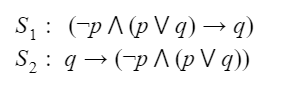Which one of the following choices is correct?
 A Both S1and S2 are tautologies. B Neither S1and S2 are tautology. C S1is not a tautology but S2is a tautology. D S1is a tautology but S2is not a tautology.
Engineering-Mathematics       Propositional-Logic       GATE 2021 CS-Set-1
Question 44 Explanation:

A tautology is a formula which is "always true" . That is, it is true for every assignment of truth values to its simple components.

Method 1:
S1: (~p ^ (p Vq)) →q
The implication is false only for T->F condition.
Let's consider q as false, then
(~p ^ (p Vq)) will be (~p ^ (p VF)) = (~p ^ (p)) =F.
It is always F->F which is true for implication. So there are no cases that return false, thus its always True i.e. its Tautology.

S2:

q->(~p (p Vq))

The false case for implication occurs at T->F case.
Let q=T then (~p (p Vq))  = (~p (p VT))= ~p. (It can be false for p=T).
So there is a case which yields T->F = F. Thus its not Valid or Not a Tautology.

Method 2: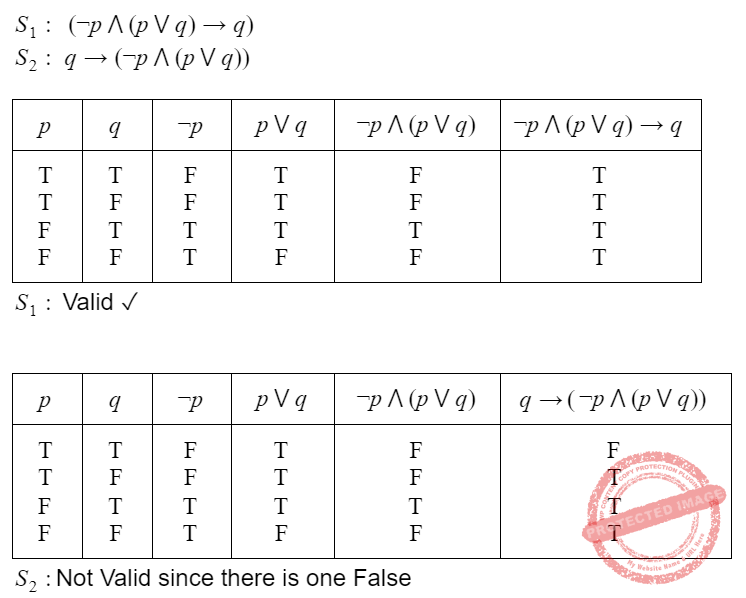Question 45
Choose the correct choice(s) regarding the following propositional logic assertion S:A S is a contradiction. B The anecdote of S is logically equivalent to the consequent of S. C S is a tautology. D S is neither a tautology nor a contradiction.
Engineering-Mathematics       Propositional-Logic       GATE 2021 CS-Set-2
Question 45 Explanation:
 Question 46
Identify the correct translation into logical notation of the following assertion. Some boys in the class are taller than all the girls Note: taller (x, y) is true if x is taller than y.
 A (∃x)(boy(x) → (∀y)(girl(y) ∧ taller(x, y))) B (∃x)(boy(x) ∧ (∀y)(girl(y) ∧ taller(x, y))) C (∃x)(boy(x) → (∀y)(girl(y) → taller(x, y))) D (∃x)(boy(x) ∧ (∀y)(girl(y) → taller(x, y)))
Engineering-Mathematics       Propositional-Logic       ISRO-2007
Question 46 Explanation:
Don't confuse with '∧' and '→'
'∧' → predicts statements are always true, no matter the value of x.
'→' → predicts there is no need of left predicate to be true always, but whenever it becomes true, then right predicate must be true.
Option D:
There exists a some boys who are taller than of all girls y.
 Question 47
Which one of the following Boolean expressions is NOT a tautology?
 A ((a → b) ∧ (b → c)) → (a → c) B (a ↔ c) → ( ¬b → (a ∧ c)) C (a ∧ b ∧ c) → (c ∨ a) D a → (b → a)
Engineering-Mathematics       Propositional-Logic       ISRO-2017 May
Question 47 Explanation:
Tautology: A universal truth in formal logic.Note: Above (A↔C) →( ¬B→(A∧C)) is not tautology.
 Question 48
The proposition (P⇒Q)⋀(Q⇒P) is a
 A tautology B contradiction C contingency D absurdity
Engineering-Mathematics       Propositional-Logic       ISRO-2017 December
Question 48 Explanation:
A proposition that is neither a tautology nor a contradiction is called a contingency.Question 49
Akash, Bharani, Chetan and Deepa are invited to a party. If Bharani and Chetan attend, then Deepa will attend too. If Bharani does not attend, then Akash will not attend. If Deepa does not attend, which of the following is true?
 A Chetan does not attend B Akash does not attend C either (a) or (b) D none of the above
Engineering-Mathematics       Propositional-Logic       CHENNAI MATHEMATICAL INSTITUTE M.Sc. / Ph.D. Programme in Computer Science (15 May 2018)
Question 49 Explanation:
If Deepa does not attend, then one of Chetan and Bharani is absent. The first case is (a). The second case is that Bharani does not attend – but it is given that Akash will not attend if Bharani does not attend. So in the second case, (b) holds. So either (a) holds or (b) holds, and hence the correct answer is (c).
 Question 50

Consider a vocabulary with only four propositions A, B, C and D. How many models are there for the following sentence ? B ∨ C

 A 10 B 12 C 15 D 16
Engineering-Mathematics       Propositional-Logic       UGC-NET CS 2018 JUNE Paper-2
Question 50 Explanation:
Here, number of models is nothing but number of TRUEs in final statement. In this proposition logic we got total 15 number of models.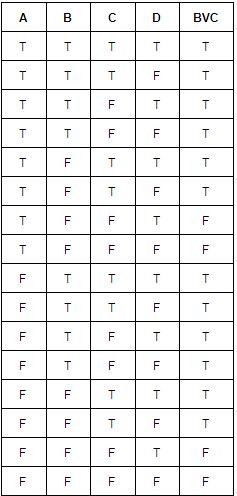Total 12 TRUE for given sentence.
 Question 51

Consider the following statements :

(a) False╞ True
(b) If α╞ (β ∧ γ) then α╞ β and α╞ γ.

Which of the following is correct with respect to the above statements ?

 A Both statement (a) and statement (b) are false. B Statement (a) is true but statement (b) is false. C Statement (a) is false but statement (b) is true. D Both statement (a) and statement (b) are true.
Engineering-Mathematics       Propositional-Logic       UGC-NET CS 2018 JUNE Paper-2
Question 51 Explanation:
(a) False╞ True is nothing but False=False|True (or) False=False V True(b) α╞ (β ∧ γ) also write αV(β ∧ γ)
α╞ β and α╞ γ also write (αVβ)∧(αVγ)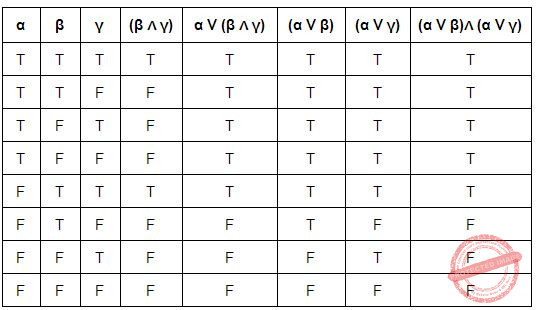Finally, we proved as LHS=RHS. αV(β ∧ γ) = (αVβ)∧(αVγ)
So, both the statements are correct.
 Question 52

Consider the following two sentences :

(a) The planning graph data structure can be used to give a better heuristic for a planning problem.
(b) Dropping negative effects from every action schema in a planning problem results in a relaxed problem.

Which of the following is correct with respect to the above sentences ?

 A Both sentence (a) and sentence (b) are false. B Both sentence (a) and sentence (b) are true. C Sentence (a) is true but sentence (b) is false. D Sentence (a) is false but sentence (b) is true.
Engineering-Mathematics       Propositional-Logic       UGC-NET CS 2018 JUNE Paper-2
Question 52 Explanation:
• Negative effects put restrictions on the action schema. When these restrictions are put into place, then the number of actions that can be taken to get to the next time step decreases because with each addition of a restriction, the actions that do not meet the restriction are filtered out.
• When these negative effects are dropped, then the number of actions increase and dropping all of the negative effects from the action schema results in a relaxed problem.
• A planning graph is a directed graph organized into levels: first a level S0 for the initial state, consisting of nodes representing each fluent that holds in S0; then a level A0 consisting of nodes for each ground action that might be applicable in S0; then alternating levels Si followed by Ai ; until we reach a termination condition.
• As a tool for generating accurate heuristics, we can view the planning graph as a relaxed problem that is efficiently solvable.
 Question 53

A knowledge base contains just one sentence, ∃x AsHighAs (x, Everest). Consider the following two sentences obtained after applying existential instantiation.

(a) AsHighAs (Everest, Everest)
(b) AsHighAs (Kilimanjaro, Everest)

Which of the following is correct with respect to the above sentences ?

 A Both sentence (a) and sentence (b) are sound conclusions. B Both sentence (a) and sentence (b) are unsound conclusions. C Sentence (a) is sound but sentence (b) is unsound. D Sentence (a) is unsound but sentence (b) is sound.
Engineering-Mathematics       Propositional-Logic       UGC-NET CS 2018 JUNE Paper-2
Question 53 Explanation:
• The ∃x AsHighAs (x, Everest) means there is one element which as highest as Everest.
• In the statement (a) AsHighAs (Everest, Everest), both are Everest then we can’t compare.
• The statement (b) AsHighAs (Kilimanjaro, Everest) means there kilimanjaro which is as highest as Everest So this valid statement.Because we are comparing Kilimanjaro with Everest.
 Question 54

The equivalence of ¬∃ x Q(x) is :

 A ∃x ¬Q(x) B ∀x ¬Q(x) C ¬∃ x ¬Q(x) D ∀x Q(x)
Engineering-Mathematics       Propositional-Logic       UGC-NET CS 2018 JUNE Paper-2
Question 54 Explanation:
We can write it as ¬∃x Q(x) = ∀x ¬Q(x).
 Question 55
If Ai = {−i, ... −2,−1, 0, 1, 2, . . . . . i} then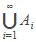is:
 A Z B Q C R D C
Engineering-Mathematics       Propositional-Logic       UGC-NET CS 2018 JUNE Paper-2
Question 55 Explanation:
• In mathematics, there are multiple number sets: the natural numbers N, the set of integers Z, all decimal numbers D, the set of rational numbers Q, the set of real numbers R and the set of complex numbers C.
Z number set:
• Z is the set of integers, ie. positive, negative or zero.
• Example: ..., -100, ..., -12, -11, -10, ..., -5, -4, -3, -2, - 1, 0, 1, 2, 3, 4, 5, ... 10, 11, 12, ..., 100, ...
• The set N is included in the set Z (because all natural numbers are part of the relative integers).
• The set A consists of Positive, negative and zero numbers.
 Question 56

Match the following in List-I and List-II, for a function f :

```       List-I                   List-II
(a) ∀x∀y(f(x)=f(y)⟶x=y)      (i) Constant
(b) ∀y∃x(f(x)=y)             (ii) Injective
(c) ∀xf(x)=k                (iii) Surjective ```

 A (a)-(i), (b)-(ii), (c)-(iii) B (a)-(iii), (b)-(ii), (c)-(i) C (a)-(ii), (b)-(i), (c)-(iii) D (a)- (ii), (b)-(iii), (c)-(i)
Engineering-Mathematics       Propositional-Logic       UGC-NET CS 2018 JUNE Paper-2
Question 56 Explanation:
• The function is injective (one-to-one) if each element of the codomain is mapped to by at most one element of the domain. An injective function is an injection. Notationally:
x, x' ∈ X, f(x) = f(x') ⇒ x = x'
Or, equivalently (using logical transposition),
x, x' ∈ X, x ≠ x' f(x) ≠ f(x')
• The function is surjective (onto) if each element of the codomain is mapped to by at least one element of the domain. (That is, the image and the codomain of the function are equal.) A surjective function is a surjection. Notationally:
∀y ∈ Y, ∃x ∈ X such that y = f(x)
A constant function is a function whose (output) value is the same for every input value.
For example, the function y(x) = 4 is a constant function because the value y(x) is 4 regardless of the input value x.
 Question 57
​ In propositional logic, which of the following is equivalent to p → q?
 A ~p → q B ~p V q C ~p V ~q D p → ~q
Engineering-Mathematics       Propositional-Logic       Nielit Scientist-C 2016 march
Question 57 Explanation:
We can use a truth table to determine if two compound propositions are logically equivalent, i.e if they always have the same truth values.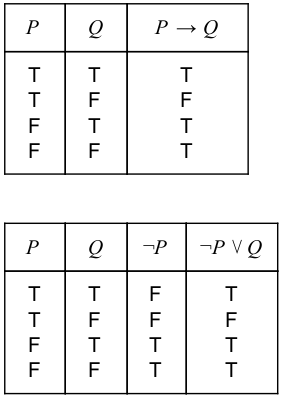Question 58

Consider the vocabulary with only four propositions A, B, C and D. How many models are there for the following sentence?

(A∨B∨C∨D)
 A 8 B 7 C 15 D 16
Engineering-Mathematics       Propositional-Logic       UGC-NET CS 2018 DEC Paper-2
Question 58 Explanation:
Here, number of models is nothing but number of TRUEs in final statement. In this proposition logic we got total 15 number of models.Question 59
Which of the following propositions is tautology.
 A (p Vq) → q B p V(q → p) C pV(p → q) D Both B) and C)
Engineering-Mathematics       Propositional-Logic       Nielit Scientist-B CS 22-07-2017
Question 59 Explanation:Question 60

Match the List 1 and List 2 and choose the correct answer from the code given below

``` LIST 1			 LIST 2
(a) Equivalence		 (i) p ⇒ q
(b) Contrapositive     	(ii) p ⇒ q : q ⇒ p
(c) Converse	       (iii) p ⇒ q : ∼q ⇒ ∼p
(d) Implication		(iv) p ⇔ q
```
Code:
 A (a)-(i), (b)-(ii), (c)-(iii), (d)-(iv) B (a)-(ii), (b)-(i),(c)-(iii), (d)-(iv) C (a)-(iv), (b)-(iii), (c)-(ii), (d)-(i) D (a)-(iii), (b)-(iv), (c)-(ii), (d)-(i)
Engineering-Mathematics       Propositional-Logic       UGC-NET CS 2018 DEC Paper-2
Question 60 Explanation:
Propositions r and s are logically equivalent if the statement r ↔ s is a tautology.
∼q⇒ ∼p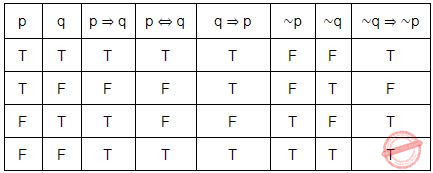According to above table,
equivalence means p ⇔ q
Contrapositive means p⇒ q : ∼q⇒ ∼p
Converse means p ⇒ q : q ⇒ p
Implication means p ⇔ q
 Question 61
​ Which of the following is FALSE?
Read ⋀ as AND, ⋁ as OR, ~ as NOT, → as one way implication and ⬌ as two way implication?
 A ((x→ y)⋀ x)->y B ((~x→ y)⋀(~x⋀~y)) → x C (x→ (x ⋁ y)) D ((x ⋁ y) ⬌ (~x ⋁ ~y))
Engineering-Mathematics       Propositional-Logic       Nielit Scientist-C 2016 march
Question 61 Explanation:Question 62
Which of the following is/are tautology?
 A a V b → b ⋀ c B a ⋀ b → b V c C a V b → (b → c) D a → b → (b → c)
Engineering-Mathematics       Propositional-Logic       NieLit STA 2016 March 2016
Question 62 Explanation:Question 63

In mathematical logic, which of the following are statements ?

(i)  There will be snow in January
(ii)  What is the time now ?
(iii)  Today is Sunday
(iv)  You must study Discrete Mathematics.

Choose the correct answer from the code given below :

Code :
 A (iii) and (iv) B (i) and (ii) C (i) and (iii) D (ii) and (iv)
Engineering-Mathematics       Propositional-Logic       UGC-NET CS 2018 DEC Paper-2
Question 63 Explanation:
In mathematical logic, the term statement is variously understood to mean either:
(a) a meaningful declarative sentence that is true or false, or
(b) the assertion that is made by a true or false declarative sentence.
From the above four statements, statement 2 and 4 wont give meaning like true or false answers and statements 1 and 3 will give either true or false answers.
 Question 64

Consider the statements below :

`“There is a country that borders both India and Nepal.“`

Which of the following represents the above sentence correctly ?

 A ∃c Border(Country(c), India ∧ Nepal) B ∃c Country(c) ∧ Border(c, India) ∧ Border(c, Nepal) C [∃c Country(c)] ⇒ [Border(c,India) ∧ Border(c, Nepal)] D ∃c Country(c) ⇒ [ Border(c, India) ∧ Border(c, Nepal)]
Engineering-Mathematics       Propositional-Logic       UGC-NET CS 2018 DEC Paper-2
Question 64 Explanation:
→ ∃c Country(c) which represents there is one country “c”
→ Border(c, India) which represents border between c and india
→ Border(c, Nepal) which represents border between c and Nepal
→ “∧” represents both
Option-2 represents the sentence “There is a country that borders both India and Nepal".
 Question 65
(PVQ) ​ ⋀ ​ (P → R) ​ ⋀ ​ (Q → R) is equivalent to
 A P B Q C R D True≅T
Engineering-Mathematics       Propositional-Logic       NieLit STA 2016 March 2016
Question 65 Explanation:Question 66
Which of the following statements is false?
 A (P⋀Q)V(~P⋀Q)V(P⋀~Q) is equal to ~Q⋀~P B (P⋀Q)V(~P⋀Q)V(P⋀~Q) is equal to QVP C (P⋀Q)V(~P⋀Q)V(P⋀~Q) is equal to QV(P⋀~q) D (P⋀Q)V(~P⋀Q)V(P⋀~Q) is equal to PV(Q⋀~p)
Engineering-Mathematics       Propositional-Logic       Nielit Scientist-B IT 22-07-2017
Question 66 Explanation:
One simple method is, by using truth table we can find the statement is true or not.The last two columns of the above table are different. So option A is false.
 Question 67

Let P, Q and R be three atomic prepositional assertions, and

X : (P ∨ Q) → R
Y : (P → R) ∨ (Q → R)

Which one of the following is a tautology?

 A X → Y B Y → X C X ≣ Y D ~Y → X
Engineering-Mathematics       Propositional-Logic       JT(IT) 2018 PART-B Computer Science
Question 67 Explanation:Question 68

Which of the given options is the logical translation of the following statement, where F(x) and P(x) express the terms friend and perfect, respectively?

“None of my friends are perfect”?

 A ∃x(~F(x)∧P(x)) B ∃x(F(x)∧ ~P(x)) C ~∃x(F(x)∧P(x)) D ∃x(~F(x)∧~P(x))
Engineering-Mathematics       Propositional-Logic       JT(IT) 2018 PART-B Computer Science
Question 68 Explanation:
F(x) represents x is friend.
P(x) represents x is perfect.
Given statement is “None of my friends are perfect”.
F(x)∧P(x) ---> X is both Friend and perfect.
~∃x ----> There is exist no one.
We can also write the above statement as follows
∀x[F(x) ⟹ ¬P(x)]
≡ ∀x[¬F(x) ∨ ¬P(x)]
≡∀x¬[F(x) ∧ P(x)]
≡¬∃x[F(x) ∧ P(x)]
 Question 69

Which of the following statements are logically equivalent?

(i) ∀x(P(x))
(ii) ~∃x(P(x))
(iii) ~∃x(~P(x))
(iv) ∃x(~P(x))
 A Only (ii) and (iv) B Only (ii) and (iii) C Only (i) and (iv) D Only (i) and (ii)
Engineering-Mathematics       Propositional-Logic       JT(IT) 2018 PART-B Computer Science
Question 69 Explanation:
∀x(P(x)) = ~∃x(~P(x))
 Question 70

In algebra of logic, the conjunction of two tautologies is:

 A Contradiction B Tautology C Negation D Disjunction
Engineering-Mathematics       Propositional-Logic       JT(IT) 2016 PART-B Computer Science
Question 70 Explanation:
Some properties are tautologies:
1. The negation of a contradiction is a tautology.
2. The disjunction of two contingencies can be a tautology.
3. The conjunction of two tautologies is a tautology.
 Question 71
Let P and Q be two propositions, ¬ (P ↔ Q) is equivalent to:
(I) P ↔ ¬ Q
(II) ¬ P ↔ Q
(III) ¬ P ↔ ¬ Q
(IV) Q → P
 A Only (I) and (II) B Only (II) and (III) C Only (III) and (IV) D None of the above
Engineering-Mathematics       Propositional-Logic       UGC NET CS 2017 Nov- paper-2
Question 71 Explanation: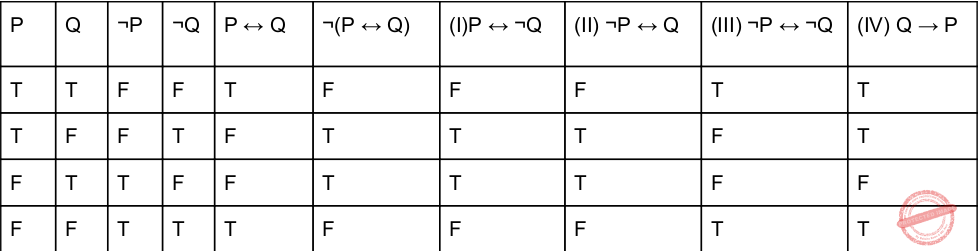So, (I) and (Ii) are TRUE.
 Question 72
Negation of the proposition ∃x H(x) is:
 A ∃x ⌐H(x) B ∀x ⌐H(x) C ∀x H(x) D ⌐x H(x)
Engineering-Mathematics       Propositional-Logic       UGC NET CS 2017 Nov- paper-2
Question 72 Explanation:
∃x H(x) = ∀x ⌐H(x)
 Question 73
Match the following :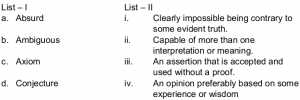A a-i, b-ii, c-iii, d-iv B a-i, b-iii, c-iv, d-ii C a-ii, b-iii, c-iv, d-i D a-ii, b-ii, c-iii, d-iv
Artificial-intelligence       Propositional-Logic       UGC NET CS 2017 Jan -paper-2
Question 73 Explanation:
Absurd→ Clearly impossible being contrary to some evident truth.
Ambiguous→ Capable of more than one interpretation or meaning.
Axiom→ An assertion that is accepted and used without a proof.
Conjecture→ An opinion preferably based on some experience or wisdom
 Question 74
In propositional logic if (P → Q) ∧ (R → S) and (P ∨ R) are two premises such thatA P ∨ R B P ∨ S C Q ∨ R D Q ∨ S E None of These
Engineering-Mathematics       Propositional-Logic       UGC NET CS 2017 Jan -paper-2
Question 74 Explanation:
Option-A: Let P be TRUE and R be false, then the conclusion PVR will be TRUE. Now if we make Q as false then premises (P→Q)∧(R→ S)will be false because P→ Q is false. Hence this option is not correct.
Option-B: Let P be TRUE and S be false then the conclusion PVS is TRUE. Now, if we make R as TRUE then the premises (P→ Q)∧(R→ S) will be false because (R→ S) will be false. Hence this option is not correct.
Option-C: Let Q be false, R be TRUE then conclusion QVR will be TRUE. Now if we make S as FALSE then Premises (P→ Q)∧(R→ S) will be FALSE because (R→ S) will be false. Hence, this option is not correct.
Option-D: Let Q be TRUE and S be FALSE then conclusion QVS will be TRUE. Now if we make R as TRUE then premises (P→ Q)∧(R→ S) will be FALSE because (R→ S) will be false.
Therefore None of the given options are correct.
Note: As per UGC NET key, given option D as correct answer.
 Question 75
Let P(m, n) be the statement “m divides n” where the Universe of discourse for both the variables is the set of positive integers. Determine the truth values of the following propositions.
(a)∃m ∀n P(m, n)
(b)∀n P(1, n)
(c) ∀m ∀n P(m, n)
 A (a) - True; (b) - True; (c) - False B (a) - True; (b) - False; (c) - False C (a) - False; (b) - False; (c) - False D (a) - True; (b) - True; (c) - True
Artificial-intelligence       Propositional-Logic       UGC NET CS 2015 Dec- paper-2
Question 75 Explanation:
Given P(m,n) ="m divides n"
Statement-A is ∃m ∀n P(m, n). Here, there exists some positive integer which divides every positive integer. It is true because there is positive integer 1 which divides every positive integer.
Statement-B is ∀n P(1, n). Here, 1 divided every positive integer. It is true.
Statement-C is ∀m ∀n P(m, n). Here, every positive integer divided every positive integer. It is false.
 Question 76
Match the following terms:A (i)(ii)(iii)(iv) B (ii)(iii)(i)(iv) C (iii)(ii)(iv)(i) D (iv)(iii)(ii)(i)
Engineering-Mathematics       Propositional-Logic       UGC NET CS 2015 Dec- paper-2
Question 76 Explanation:
→ Vacuous proof is a proof that the implication p → q is true based on the fact that p is false.
→ Trivial proof is a proof that the implication p → q is true based on the fact that q is true.
→ Direct proof is a proof that the implication p → q is true that proceeds by showing that q must be true when p is true.
→ Indirect proof is a proof that the implication p → q is true that proceeds by showing that p must be false when q is false.
 Question 77
Consider the compound propositions given below as:
(a)p ∨ ~(p ∧ q)
(b)(p ∧ ~q) ∨ ~(p ∧ q)
(c)p ∧ (q ∨ r)
Which of the above propositions are tautologies?
 A (a) and (c) B (b) and (c) C (a) and (b) D only (a)
Engineering-Mathematics       Propositional-Logic       UGC NET CS 2015 Dec- paper-2
Question 77 Explanation: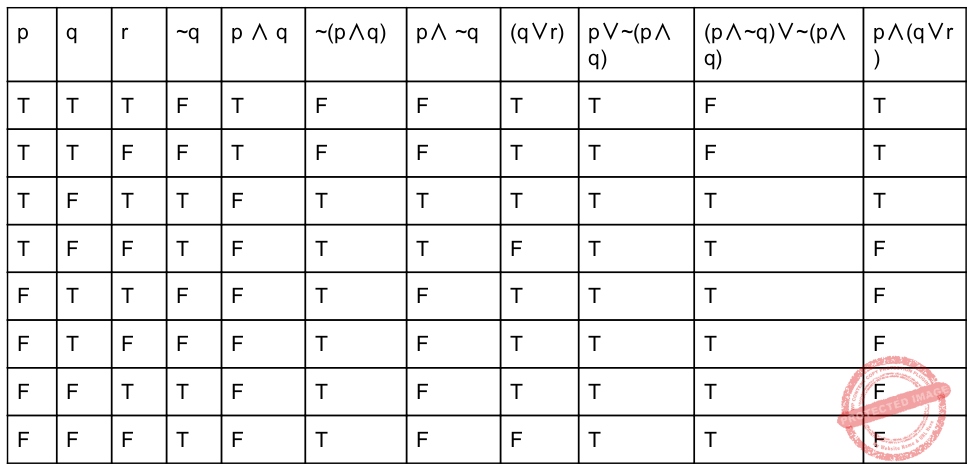Question 78
“If my computations are correct and I pay the electric bill, then I will run out of money. If I don’t pay the electric bill, the power will be turned off. Therefore, if I don’t run out of money and the power is still on, then my computations are incorrect.” Convert this argument into logical notations using the variables c, b, r, p for propositions of computations, electric bills, out of money and the power respectively. (Where ¬ means NOT)
 A if (c Λ b)→r and ¬b→p, then (¬r Λ p)→¬c B if (c ∨ b)→r and ¬b→¬p, then (r Λ p)→c C if (c Λ b)→r and ¬p→b, then (¬r ∨ p)→¬c D if (c ∨ b)→r and ¬b→¬p, then (¬r Λ p)→¬c
Engineering-Mathematics       Propositional-Logic       UGC NET CS 2015 Jun- paper-2
Question 78 Explanation:
We can represent ,
“c” for my computations are correct
“b” for I pay the electric bill.
“r” for I will run out of money
“p” for the power is on.
(c Λ b) means my computations are correct and I pay the electric bill.
(¬r Λ p) means I don’t run out of money and the power is still on.
According to the statement , the option -(A) is correct.
 Question 79
Match the following:A (a)-(i), (b)-(ii), (c)-(iii), (d)-(iv) B (a)-(ii), (b)-(iii), (c)-(i), (d)-(iv) C (a)-(iii), (b)-(ii), (c)-(iv), (d)-(i) D (a)-(iv), (b)-(ii), (c)-(iii), (d)-(i)
Engineering-Mathematics       Propositional-Logic       UGC NET CS 2015 Jun- paper-2
Question 79 Explanation:
→ In logic, contraposition is an inference that says that a conditional statement is logically equivalent to its contrapositive. The contrapositive of the statement has its antecedent and consequent inverted and flipped: the contrapositive of P → Q is thus as ~Q → ~P.
→ The equivalence relation translates verbally into "if and only if" and is symbolized by a double-lined, double arrow pointing to the left and right (↔). If A and B represent statements, then A ↔ B means "A if and only if B."
→ The exportation rule is a rule in logic which states that "if (P and Q), then R" is equivalent to "if P then (if Q then R)".
→ The exportation rule may be formally stated as: (P ∧ Q) → R is equivalent to P → (Q →R)
→ A mode of argumentation or a form of argument in which a proposition is disproven by following its implications logically to an absurd conclusion. Arguments that use universals such as, “always”, “never”, “everyone”, “nobody”, etc., are prone to being reduced to absurd conclusions. The fallacy is in the argument that could be reduced to absurdity -- so in essence, reductio ad absurdum is a technique to expose the fallacy.
 Question 80
AVA=A is called :
 A Identity law B De Morgan’​ s law C Idempotent law D Complement law
Engineering-Mathematics       Propositional-Logic       UGC NET CS 2004 Dec-Paper-2
Question 80 Explanation:
→ ​ De Morgan’s Laws:
(i). (A ​ V ​ B)’ = A' ​ ∧ ​ B'
(ii). (A ​ ∧ ​ B)’ = A' ​ V ​ B'
→ ​ Identity Law​ :
(i). 1 AND A = A
(ii). 0 OR A = A
→ ​ Complement law:
(i). A AND A'=1
(ii). A OR A'=0
→ ​ Idempotent law:
The idempotence in the context of elements of algebras that remain invariant when raised to a positive integer power, and literally means "(the quality of having) the same power", from idem + potence (same + power).
(i). A V A=A
(ii). A ∧ A=A
According to boolean algebraQuestion 81
If the proposition ​ ¬ ​ P ​ → ​ Q is true, then the truth value of the proportion ​ ¬ ​ PV (P ​ → ​ Q) is:
 A True B Multi - Valued C Flase D Can not determined
Engineering-Mathematics       Propositional-Logic       UGC NET CS 2005 Dec-Paper-2
Question 81 Explanation:
We can also write (¬p → q) into (p ∨ q)Here, we can minimize the boolean form into
¬p ∨ (p → q)
= ¬p ∨ (¬p ∨ q)
= ¬p ∨ q
In question, they are not discussed about the truth values of p, it implies that ¬p ∨ q also be True or False. So, we cannot be determined.
 Question 82
The proposition ~q ∨ p is equivalent to :
 A Not given any option B Not given any option C Not given any option D Not given any option E ~q ∨ p ≣ q → p
Engineering-Mathematics       Propositional-Logic       UGC NET CS 2006 Dec-paper-2
Question 82 Explanation:
Options are not given. Excluded for evaluation.
~q ∨ p ≣ q → p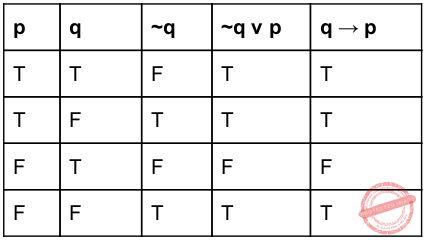Question 83
The preposition( p→q) ∧ (~q ∨ p) is equivalent to :
 A q →p B p→ q C (q →p)∨(p→ q) D (p →q)∨(q→ p) E None of the above
Engineering-Mathematics       Propositional-Logic       UGC NET CS 2006 June-Paper-2
Question 83 Explanation:Question 84
​Consider the vocabulary with only four propositions A,B,C and D. How many models are there for the following sentence?
( ​ ⌐ ​ A ∨​ ⌐ ​ B ∨​ ⌐ ​ C ∨​ ⌐ ​ D)
 A 8 B 7 C 15 D 16
Engineering-Mathematics       Propositional-Logic       UGC NET CS 2018-DEC Paper-2
Question 84 Explanation:
Here, number of models is nothing but number of TRUEs in final statement. In this proposition logic we got total 15 number of models.Question 85
The notation ∃!xP(x) denotes the proposition “there exists a unique x such that P(x) is true”.
Give the truth values of the following statements :
I. ∃!xP(x) → ∃xP(x)
II. ∃!x ¬ P(x) → ¬∀xP(x)
 A Both I & II are true. B Both I & II are false. C I – false, II – true D I – true, II – false
Engineering-Mathematics       Propositional-Logic       UGC NET CS 2014 June-paper-2
Question 85 Explanation:
I: If there exists a unique x with P(x) true, then there exist an x with P(x) true. This is TRUE as exactly 1 is a subset of at least one.
II: If there exists a unique x with P(x) false, then there does not exist an x with P(x) true. This is FALSE (contradiction) as all except one x can are having P(x) true.
 Question 86
Give a compound proposition involving propositions p, q and r that is true when exactly two of p, q and r are true and is false otherwise.
 A (p∨q∧¬r) ∧ (p∧¬q∧r) ∧ (¬p∧q∧r) B (p∧q∧¬r) ∧ (p∨q∧¬r) ∧ (¬p∧q∧r) C (p∧q∧¬r) ∨ (p∧¬q∧r) ∧ (¬p∧q∧r) D (p∧q∧¬r) ∨ (p∧¬q∧r) ∨ (¬p∧q∧r)
Engineering-Mathematics       Propositional-Logic       UGC NET CS 2014 June-paper-2
Question 86 Explanation:Here, As per the given question, option-D is correct answer.
 Question 87
In mathematical logic, which of the following are statements ?
(i) There will be snow in January
(ii) What is the time now ?
(iii) Today is Sunday
(iv) You must study Discrete Mathematics.
 A (iii) and (iv) B (i) and (ii) C (i) and (iii) D (ii) and (iv)
Engineering-Mathematics       Propositional-Logic       UGC NET CS 2018-DEC Paper-2
Question 87 Explanation:
In mathematical logic, the term statement is variously understood to mean either:
(a) a meaningful declarative sentence that is true or false, or
(b) the assertion that is made by a true or false declarative sentence.
From the above four statements , statement 2 and 4 wont give meaning like true or false answers and statements 1 and 3 will give either true or false answers
 Question 88
Consider the statements below :
“ There is a country that borders both India and Nepal. “
Which of the following represents the above sentence correctly ?
 A ∃c Border(Country(c), India ∧ Nepal) B ∃c Country(c) ∧ Border(c, India) ∧ Border(c, Nepal) C [∃c Country(c)] ⇒ [Border(c,India) ∧ Border(c, Nepal)] D ∃c Country(c) ⇒ [ Border(c, India) ∧ Border(c, Nepal)]
Engineering-Mathematics       Propositional-Logic       UGC NET CS 2018-DEC Paper-2
Question 88 Explanation:
→ ∃c Country(c) which represents there is one country “c”
→ Border(c, India) which represents border between c and india
→ Border(c, Nepal) which represents border between c and Nepal
→ “∧” represents both
Option-2 represents the sentence “ There is a country that borders both India and Nepal.
 Question 89
The quantification ∃!x P(x) denotes the proposition “There exists a unique x such that P(x) is true”,express the quantification using universal and existential quantification's and logical operators :
 A ∃x P(x) ∨ ∀x∀y ((P(x) ∨ P(y)) → x = y) B ∀x P(x) ∧ ∀x∀y ((P(x) ∨ P(y) → x = y) C ∃x P(x) ∧ ∀x∀y ((P(x) ∧ P(y)) → x = y) D ∃x P(x) ∧ ∃x∃y ((P(x) ∨ P(y)) → x = y)
Engineering-Mathematics       Propositional-Logic       UGC NET CS 2013 Sep-paper-2
Question 89 Explanation:
According to the given data, the possible solution is ∃x P(x) ∧ ∀x∀y ((P(x) ∧ P(y)) → x = y)
 Question 90
Let P(m, n) be the statement “m divides n” where the universe of discourse for both the variables is the set of positive integers. Determine the truth values of each of the following propositions :
I. ∀m ∀n P(m, n),
II. ∃m ∀n P(m, n)
 A Both I and II are true B Both I and II are false C I – false & II – true D I – true & II – false
Engineering-Mathematics       Propositional-Logic       UGC NET CS 2013 Dec-paper-2
 Question 91
The proposition ~qvp is equivalent to
 A p → q B q → p C p ↔ q D p ∨ q
Engineering-Mathematics       Propositional-Logic       UGC NET CS 2011 Dec-Paper-2
Question 91 Explanation: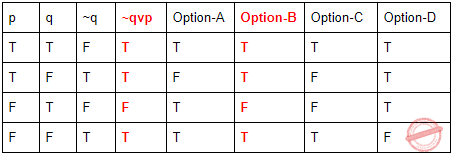Hence Option-B is correct answer
 Question 92
The proposition ~p ∨ q is equivalent to
 A p → q B q → p C p ↔ q D p ∨ q
Engineering-Mathematics       Propositional-Logic       UGC NET CS 2011 June-Paper-2
Question 92 Explanation: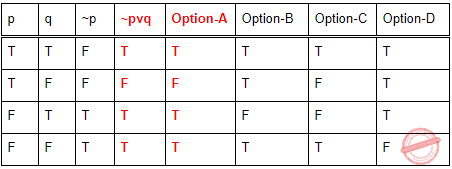Question 93
Which of the following shall be a compound proposition involving the propositions p, q and r, that is true when exactly two of the p, q and r are true and is false otherwise
 A (p ∨ q ∧ ⌐r) ∨ ( p ∧ q ∧ r) ∧ (⌐p ∧ q ∨ r) B (p ∧ q ∨ r) ∧ ( p ∧ q ∧ r) ∨ (⌐q ∧ ⌐p∧ ⌐r) C (p ∧ q ∧⌐ r) ∨ ( p ∧ ⌐q ∧ r) ∨ (⌐ p ∧ q ∧ r) D (p ∨ r ∧ q) ∨ ( p ∧ q ∧ r) ∨ (⌐p ∧ q ∧ r)
Engineering-Mathematics       Propositional-Logic       UGC NET CS 2013 June-paper-2
Question 93 Explanation:
From the question, the propositions consists of exactly two of the variables should true and final proposition should be true.
Only option C consists of exactly two of the variables are true and all remaining variables deviating this rule.
In this proposition, (p ∧ q ∧⌐ r) ∨ ( p ∧ ⌐q ∧ r) ∨ (⌐ p ∧ q ∧ r)
(p ∧ q ∧⌐ r) → p and q are true
( p ∧ ⌐q ∧ r) → p and r are true
(⌐ p ∧ q ∧ r) → q and r are true
Finally the proposition consists of “V” operation , so the entire proposition is true.
 Question 94
The truth value of the statements : ∃!xP(x) → ∃xP(x) and ∃!x ⌐P(x) →⌐∀xP(x), (where the notation ∃!xP(x) denotes the proposition “There exists a unique x such that P(x) is true”) are :
 A True and False B False and True C False and False D True and True
Engineering-Mathematics       Propositional-Logic       UGC NET CS 2013 June-paper-2
Question 94 Explanation:
From the given question, → The symbol ∃ is call the existential quantifier and represents the phrase “there exists” or “for some”. The existential quantification of P(x) is the statement “P(x) for some values x in the universe”, or equivalently, “There exists a value for x such that P(x) is true”, which is written ∃xP(x). So the statement ∃!xP(x) → ∃xP(x) is true.
→ If P(x) is true for at least one element in the domain, then ∃xP(x) is true. Otherwise it is false.
→ Accordingly DeMorgan’s laws for quantifiers:the following statements are true.
¬∀xP(x) ≡ ∃x¬P(x)
¬∃xP(x) ≡ ∀x¬P(x) then the statement is ∃!x ⌐P(x) →⌐∀xP(x) is true.
 Question 95
The propositional formula given by the tree :A ∧ ∨ x2 ∨ x1¬x1¬x1 B (x2∨ ¬x2) ∧ (x1 ∨ x2) C (¬x1∨ x2) ∧ (¬x1∨ x2) D None
Engineering-Mathematics       Propositional-Logic       UGC NET CS 2009-June-Paper-2
Question 95 Explanation:
By default we are using inorder traversal to evaluate it.
Inorder traversal starts from left,root and right.
Inorder traversal = (¬x1∨ x2) ∧ (¬x1∨ x2)
 Question 96
Twin primes are pairs of numbers p and p+2 such that both are primes—for instance, 5 and 7, 11 and 13, 41 and 43. The Twin Prime Conjecture says that there are infinitely many twin primes. Let TwinPrime(n) be a predicate that is true if n and n+2 are twin primes. Which of the following formulas, interpreted over positive integers, expresses that there are only finitely many twin primes?
 A ∀m. ∃n. m ≤ n and not(TwinPrime(n)) B ∃m. ∀n. n ≤ m implies TwinPrime(n) C ∀m. ∃n. n ≤ m and TwinPrime(n) D ∃m. ∀n. TwinPrime(n) implies n ≤ m
Engineering-Mathematics       Propositional-Logic       CHENNAI MATHEMATICAL INSTITUTE(M.Sc. / Ph.D. Programme in Computer Science) 18May 2015
Question 96 Explanation:
This says that there is a bound, m, such that any twin prime is below m. In other words, that there only finitely many twin primes.
 Question 97
You arrive at a snack bar and you can’t decide whether to order a lime juice or a lassi. You decide to throw a fair 6-sided die to make the choice, as follows. • If you throw 2 or 6 you order a lime juice. • If you throw a 4, you order a lassi. • Otherwise, you throw the die again and follow the same algorithm. What is the probability that you end up ordering a lime juice?
 A 1/3 B 1/2 C 2/3 D 3/4
Engineering-Mathematics       Propositional-Logic       CHENNAI MATHEMATICAL INSTITUTE(M.Sc. / Ph.D. Programme in Computer Science) 18May 2015
Question 97 Explanation:
The probability of getting a lime juice on any throw is 1/3 . At any stage, the probability that you will throw the dice again is 1/2 . Hence the probability that you throw the dice i times is 1/(2 i−1).Thus the overall probability of getting a lime juice isQuestion 98
An example of a tautology is :
 A x v y B x v (~y) C x v (~x) D (x=>y) v (x<=y)
Engineering-Mathematics       Propositional-Logic       UGC NET CS 2008-june-Paper-2
Question 98 Explanation:Question 99
Four siblings go shopping with their father. If Abhay gets shoes, then Asha does not get a necklace. If Arun gets a T-shirt, then Aditi gets bangles. If Abhay does not get shoes or Aditi gets bangles, the mother will be happy. Which of the following is true?
 A If the mother is happy, then Aditi got bangles. B If Aditi got bangles, then Abhay got shoes. C If the mother is not happy, then Asha did not get a necklace and Arun did not get a T-shirt. D None of the above.
Engineering-Mathematics       Propositional-Logic       CHENNAI MATHEMATICAL INSTITUTE M.Sc. / Ph.D. Programme in Computer Science (18 May 2017)
Question 99 Explanation:
Let p denote that Asha get a necklace, q denote that Abhay gets shoes, r denote that Arun gets a T-shirt, s denote that Aditi gets bangles and w denote that mother is happy. The question is equivalent to the formula φ : q → ¬p ∧ r → s ∧ (¬q ∨ s) → w. A formula ψ is a valid implication iff every truth assignment to p, q, r, s, w that makes φ true also makes ψ true. Option (a) is the formula ψ a : w → s, option (b) is the formula ψ b : s → q and option (c) is the formula ψ c : ¬w → (¬p ∧ ¬r). Neither ψ a nor ψ b are valid implications but ψ c is.
 Question 100
A WFF that is equivalent to the WFF x=>y is :
 A y=> x B ~ y=> x C ~ y=> ~ x D y=> ~ x
Engineering-Mathematics       Propositional-Logic       UGC NET CS 2007-Dec-Paper-2
Question 100 Explanation: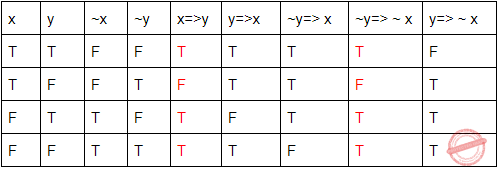Question 101
The symbol | reads as “divides”, and -| as “does not divide”. For instance, 2 | 6 and 2 -| 5 are both true. Consider the following statements: (i) There exists a positive integer a such that (2 | (a 3 − 1)) and (2 | a). (ii) There exists a positive integer b such that 6 -| (b 3 − b). What can you say about these statements?
 A Only (i) is true. B Only (ii) is true. C Both (i) and (ii) are true. D Neither (i) nor (ii) is true.
Engineering-Mathematics       Propositional-Logic       CHENNAI MATHEMATICAL INSTITUTE M.Sc. / Ph.D. Programme in Computer Science (18 May 2016)
Question 101 Explanation:
(i) is false. Note that (a 3 − 1) = (a − 1)(a 2 + a + 1), and hence 2 | a implies that both a − 1 and a 2 + a + 1 are odd, and hence so is their product. Thus 2 -| (a 3 − 1).
(ii) is false too. Note that (b 3 − b) = (b − 1)b(b + 1), and at least one of these factors is even and one is divisible by 3. Hence 6 | (b 3 − b).
 Question 102
An advertisement for a tennis magazine states, “If I’m not playing tennis, I’m watching tennis. And if I’m not watching tennis, I’m reading about tennis.” We can assume that the speaker can do at most one of these activities at a time. What is the speaker doing?
 A Playing tennis. B Watching tennis. C Reading about tennis. D None of the above.
Engineering-Mathematics       Propositional-Logic       CHENNAI MATHEMATICAL INSTITUTE M.Sc. / Ph.D. Programme in Computer Science (18 May 2016)
Question 102 Explanation:
If he is not watching tennis, then he is reading about tennis. Which means he is not playing tennis, which implies that he is watching tennis. Thus, the assumption that he is not watching tennis leads to a contradiction. Therefore he is watching tennis.
 Question 103
A company is due to send a shipment to a client and the CEO has resigned. To select a new CEO, some candidates have been interviewed. One of them will be chosen through a vote. If the workers union resort to a strike and the candidates have to be interviewed again, then the shipment deadline will be missed. If there are more abstainers than voters in the vote to choose the new CEO, then the candidates have to be interviewed again. Suppose that the shipment was sent on time. Which of the following is a valid conclusion?
 A The workers union did not resort to a strike. B The number of voters was more than the number of abstainers. C (a) or (b). D If the workers union resorted to a strike, then the number of voters was greater than or equal to the number of abstainers.
Engineering-Mathematics       Propositional-Logic       CHENNAI MATHEMATICAL INSTITUTE - M.Sc. / Ph.D. Programme in Computer Science ( 15 May 2014 )
Question 103 Explanation:
Let p, q, r, s denote the following assertions:
p : abstainers > voters
q : interview repeated
r : workers strike
s : shipment missed
Premises: p IMPLIES q, (q AND r) IMPLY s
Since it is given that shipment was sent on time, s is false.
By contrapositive, (NOT q) OR (NOT r) and (NOT q) IMPLIES (NOT p) are valid conclusions.
(a) says (NOT r), which not valid since since r could be true when s is false.
(b) says voters > abstainers, which is not valid since even if voters ≤ abstainers, s could be false.
(c) says (NOT r) OR (voters > abstainers), which not valid since we can have both r and voters ≤ abstainers true and still have s false.
(d) says r IMPLIES (voters ≥ abstainers). This is valid since (NOT q) OR (NOT r) is valid, which means r is false or q is false. If r is true, then q is false and hence p is false, which means abstainers ≤ voters.
 Question 104
Let P, Q, R and S be Propositions. Assume that the equivalences P ⇔ (Q ∨ ¬ Q) and      Q ⇔ R hold. Then the truth value of the formula (P ∧ Q) ⇒ ((P ∧ R) ∨ S) is always:
 A True B False C Same as truth table of Q D Same as truth table of S
Engineering-Mathematics       Propositional-Logic       UGC NET CS 2017 Nov- paper-3
Question 104 Explanation:
Given data,
-- Equivalence condition(Both RHS and LHS should be TRUE)= P ⇔ (Q V ~Q)
-- Holding condition(Both RHS and LHS may TRUE/FALSE)= Q ⇔ RQuestion 105
“If X, then Y unless Z” is represented by which of the following formulae in propositional logic?
 A (X ∧ Y) → ¬ Z B (X ∧ ¬ Z) → Y C X → (Y ∧ ¬ Z) D Y → (X ∧ ¬ Z)
Engineering-Mathematics       Propositional-Logic       UGC NET CS 2017 Nov- paper-3
Question 105 Explanation:
"If X then Y unless Z" ⇒ ¬Z → (X→Y)
⇒ Z ∨ ¬X ∨ Y
⇒ ¬X ∨ Z ∨ Y
Option B: (X ∧ ¬Z) → Y
= ¬(X ∧ ¬Z ) ∨ Y
= ¬X ∨ Z ∨ Y
Hence, option (B) is correct.
 Question 106
Consider the following two well-formed formulas in propositional logic.
F1 : P ⇒ ¬ P
F2 : (P ⇒ ¬ P) ∨ (¬ P ⇒ P)
Which of the following statements is correct?
 A F1 is Satisfiable, F2 is valid B F1 is unsatisfiable, F2 is Satisfiable C F1 is unsatisfiable, F2 is valid D F1 and F2 both are Satisfiable
Engineering-Mathematics       Propositional-Logic       UGC NET CS 2017 Nov- paper-3
Question 106 Explanation:Question 107
The first order logic (FOL) statement ((R ∨ Q) ∧ (P ∨ ¬Q)) is equivalent to which of the following ?
 A ((R ∨ ¬Q) ∧ (P ∨ ¬Q) ∧ (R ∨ P)) B ((R ∨ Q) ∧ (P ∨ ¬Q) ∧ (R ∨ P)) C ((R ∨ Q) ∧ (P ∨ ¬Q) ∧ (R ∨ ¬P)) D ((R ∨ Q) ∧ (P ∨ ¬Q) ∧ ( ¬R ∨ P))
Engineering-Mathematics       Propositional-Logic       UGC NET CS 2017 Jan- paper-3
Question 107 Explanation:
Method-1: Boolean simplification
((R ∨ Q) ∧ (P ∨ Q’))
(R∨Q)∧(P∨Q’)∧(R∨P)
(PR ∨ Q’R ∨ PQ) ∧ (R∨P)
PR ∨ RQ’ ∨ PQR ∨ PR ∨ PRQ’ ∨ PQ
(RQ’∨PQ’R)∨(PQR∨PQ)∨PR
RQ’ ∨ PQ ∨ PR
((R ∨ Q) ∧ (P ∨ Q’) ∧ (R ∨ P))
Method-2: Using Truth table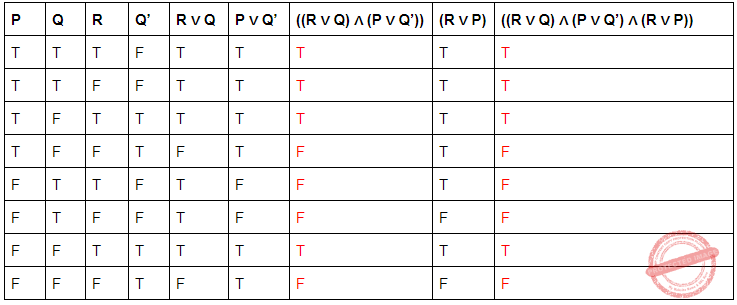Question 108
Let ν(x) mean x is a vegetarian, m(y) for y is meat, and e(x, y) for x eats y. Based on these, consider the following sentences :
I. ∀x ν(x ) ⇔ (∀y e(x, y) ⇒ ¬m(y))
II. ∀x ν(x ) ⇔ (¬(∃ym(y) ∧e(x, y)))
III. ∀x (∃y m(y) ∧e(x, y)) ⇔ ¬ν(x)
One can determine that
 A Only I and II are equivalent sentences B Only II and III are equivalent sentences. C Only I and III are equivalent sentence D I, II, and III are equivalent sentences.
Engineering-Mathematics       Propositional-Logic       UGC NET CS 2016 Aug- paper-3
 Question 109
Consider the following logical inferences :
I1 : If it is Sunday then school will not open.
The school was open.
Inference : It was not Sunday.
I2 : If it is Sunday then school will not open.
It was not Sunday.
Inference : The school was open.
Which of the following is correct ?
 A Both I1 and I2 are correct inferences. B I1 is correct but I2 is not a correct inference. C I1 is not correct but I2 is a correct inference. D Both I1 and I2 are not correct inferences.
Engineering-Mathematics       Propositional-Logic       UGC NET CS 2016 Aug- paper-3
 Question 110
In Propositional Logic, given P and P → Q, we can infer __________.
 A ~ Q B Q C P ∧ Q D ~ P ∧ Q
Engineering-Mathematics       Propositional-Logic       UGC NET CS 2015 Dec - paper-3
Question 110 Explanation:
Modus ponens rule / Rule of detachment: According to Modus ponens rule given P and P → Q, we can infer Q
 Question 111
Which of the following is principal conjunctive normal form for [(pVq) ∧ ~p → ~q]?
 A pV~q B pVq C ~p Vq D ~p V ~q
Engineering-Mathematics       Propositional-Logic       UGC NET June-2019 CS Paper-2
Question 111 Explanation:Question 112
Match List-I with List-II
List-I                          List-II
(a) p → q                (i) ¬(q → ¬p)
(b) p v q                  (ii) p ∧ ¬q
(c) p ∧ q                  (iii) ¬p → q
(d) ¬(p → q)            (iv) ¬p v q
Choose the correct option from those given below:
 A (a)-(ii);(b)-(iii);(c)-(i);(d)-(iv) B (a)-(ii);(b)-(i);(c)-(iii);(d)-(iv) C (a)-(iv);(b)-(i);(c)-(iii);(d)-(ii) D (a)-(iv);(b)-(iii);(c)-(i);(d)-(ii)
Engineering-Mathematics       Propositional-Logic       UGC NET June-2019 CS Paper-2
Question 112 Explanation:Question 113
The notation “⇒” denotes “implies” and “∧” denotes “and” in the following formulae.
Let X denote the formula: (b ⇒ a) ⇒ (a ⇒ b)
Let Y denote the formula: (a ⇒ b) ∧ b
Which of the following is TRUE?
 A X is satisfiable and Y is not satisfiable. B X is satisfiable and Y is a tautology. C X is not a tautology and Y is not satisfiable. D X is not a tautology and Y is satisfiable. E X is a tautology and Y is satisfiable.
Engineering-Mathematics       Propositional-Logic       TIFR PHD CS & SS 2018
 Question 114
The clausal form of the disjunctive normal form ¬A ∨ ¬B ∨ ¬C ∨ D is:
 A A ∧ B ∧ C ⇒ D B A ∨ B ∨ C ∨ D ⇒ true C A ∧ B ∧ C ∧ D ⇒ true D A ∧ B ∧ C ∧ D ⇒ false
Engineering-Mathematics       Propositional-Logic       UGC NET CS 2015 June Paper-3
Question 114 Explanation: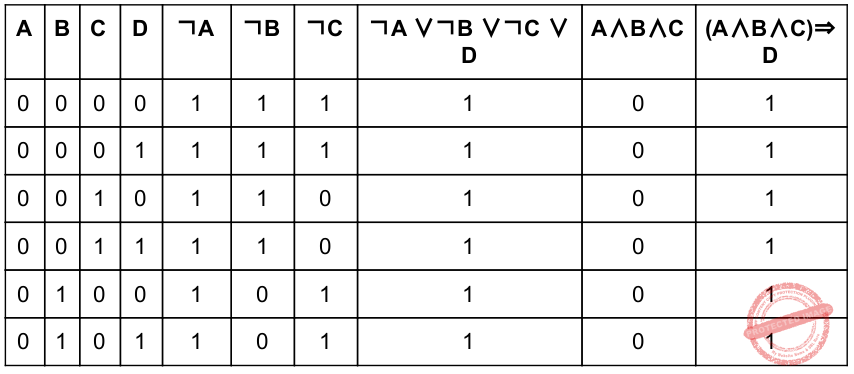Question 115
In propositional logic P ↔ Q is equivalent to (Where ~ denotes NOT):
 A ~( P ∨ Q ) ∧ ~ ( Q ∨ P ) B ( ~P ∨ Q ) ∧ (~ Q ∨ P ) C ( P ∨ Q ) ∧ ( Q ∨ P ) D ~( P ∨ Q ) → ~ ( Q ∨ P )
Engineering-Mathematics       Propositional-Logic       UGC NET CS 2015 June Paper-3
Question 115 Explanation: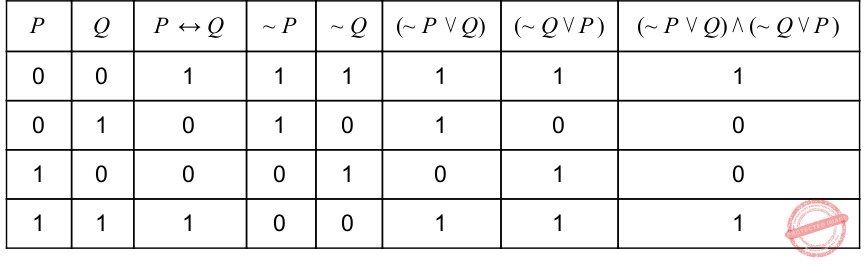Question 116

Consider the following statements: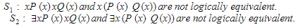Which of the following statements is/are correct?
 A Only S1 B Only S2 C Both S1 and S2 D Neither S1 nor S2
Engineering-Mathematics       Propositional-Logic       UGC-NET DEC-2019 Part-2
 Question 117
If Vinay finishes his homework and the school closes early, then he can play in the park or eat an ice cream. He will end up at the dispensary with tummy ache if he eats ice cream and plays in the park. Which of the following can be correctly inferred?,
 A If he doesn’t end up in the dispensary with tummy ache, then he did not finish his homework or the school closed late. B If he doesn’t end up in the dispensary with tummy ache, he didn’t eat ice cream and he didn’t play in the park. C Both (a) and (b) D None of the above.
Engineering-Mathematics       Propositional-Logic       CMI PHD 2022
Question 117 Explanation:
(a) is not possible, because Vinay can finish his homework, the school can close early, and he plays in the park without eating icecream. In this case he will not end up in the dispensary, but neither of the conclusions in (a) can be inferred. (b) is not possible, again because Vinay can play in the park without eating icecream, or eat icecream without playing in the park. In both cases, he does not end up in the dispensary. So one cannot infer the conjunction of the two conclusions in (b). Hence the correct answer is (d).
 Question 118
Given that
B() means “is a bear”
F() means “is a fish” and
E(a,b) means “ eats b”
Then what is the best meaning ofA Every fish is eaten by some bear B Bears eat only fish C Every bear eats fish D Only bears eat fish
Engineering-Mathematics       Propositional-Logic       ISRO CS 2020       Video-Explanation
Question 118 Explanation:
Let's begin with given quantifiers. → For any Fish x, for any y is eaten by y, then y must be a bear. → Which means only bear eats fish, option D is correct → Option C says every bear eats fish, but given that every fish eaten by bear only which is not equal given. → Option B says bear eats only fish, but bear may eat anything. → Option A says every fish eaten by some bear, but it's not a single bear, it must by any bear.
 Question 119
In first order predicate logic, ∼∀x P(x) is equivalent to Options:
 A ∼∃x P(x) B ∃x ∼P(x) C ∀x ∼P(x) D the given expression is not a well formed
Engineering-Mathematics       Propositional-Logic       APPSC-2016-DL-CS
Question 119 Explanation:
∼∀x P(x) = ∃x ∼P(x)
 Question 120
The propositional expression [(∼P∨Q)→(Q→P)] is
 A a tautology B not a tautology C contradiction D not a well-formed formula
Engineering-Mathematics       Propositional-Logic       APPSC-2016-DL-CS
Question 120 Explanation:Question 121
Which of the following predicate expressions represent the statement “None of the Students have failed in the test”?
 A ∼∃x(Student(x)∧∼Failed(x)) B ∼∀x(∼Student(x)∧∼Failed(x)) C ∀x(∼Student(x)∧FAiled(x)) D ∼∃x(Student(x)∧Failed(x))
Engineering-Mathematics       Propositional-Logic       APPSC-2016-DL-CS
 Question 122

What are you predicting by the logic:

` ∨x:€y:loyalto(x,y) `
 A Everyone is loyal to someone B Everyone is loyal to all C Everyone is not loyal to someone D Everyone is loyal
Engineering-Mathematics       Propositional-Logic       APPSC-2016-DL-CA
 Question 123

An inference rule that says, if you know x and you know that if x is true, then y is true, then you can conclude y is

 A Minmax rule B Modus Pones rule C Chain rule D None of the given options
Engineering-Mathematics       Propositional-Logic       APPSC-2016-DL-CA
Question 123 Explanation:
Modus ponen rule says that "P implies Q and P is asserted to be true, therefore Q must be true."
 Question 124

An example of a tautology is

 A x ∨ y B x ∨ (¬ y) C x ∨ (¬ x) D (x →y) ^ (x ← y)
Engineering-Mathematics       Propositional-Logic       APPSC-2016-DL-CA
Question 124 Explanation: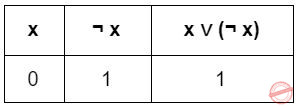Question 125

The idempotent law in the Boolean algebra says that

 A ¬ (¬ x) = x B x + x = x C x + xy = x D x(x + y) = x
Engineering-Mathematics       Propositional-Logic       APPSC-2016-DL-CA
Question 125 Explanation:
The idempotent law in the Boolean algebra says that a op a = a, where op is any operator.
 Question 126

The proposition (p→q) ^ (¬ q ∨ (p ∨ p) is equivalent to

 A q → p B p → q C (q → p) ∨ (p → q) D (p → q) ∧ (q → p)
Engineering-Mathematics       Propositional-Logic       APPSC-2016-DL-CA
Question 126 Explanation:
(p→q) ^ (¬ q ∨ (p ∨ p) = (p->q) ^ (¬ q v p) (since pvp = p)
= (p->q) ^ (q ->p) (since ¬ q v p = q->p)
 Question 127

Which of the following are tautologies?

 A ((PvQ)^Q↔Q B (Pv(P→Q))→P C ((PvQ)^P)→Q D ((PvQ)^P)→Q
Engineering-Mathematics       Propositional-Logic       APPSC-2016-DL-CA
Question 127 Explanation:
Let's check option 1,
((P v Q) ^ Q) <-> Q
Lets solve LHS,
(P v Q) ^ Q = (P ^ Q) v (Q ^ Q) = (P ^ Q) v Q = PQ + Q = Q(1+P) = Q
So LHS = RHS. Hence tautology.
Similarly check for all other options, none of the other options will get LHS = RHS.
 Question 128
________predicate calculus allows quantified variables to refer to objects in the domain of discourse and not to predicates or functions.
 A Zero-order B First-order C Second-order D High-order
Engineering-Mathematics       Propositional-Logic       UGC NET CS 2014 June-paper-3
Question 128 Explanation:
First-order predicate calculus allows quantified variables to refer to objects in the domain of discourse and not to predicates or functions.
 Question 129

Equivalent logical expression for the Well Formed Formula (WFF),

~(∀x) F[x]

is
 A ∀x (~F[x]) B ~(∃x) F[x] C ∃x (~F[x]) D ∀x F[x]
Engineering-Mathematics       Propositional-Logic       UGC NET CS 2014 Dec - paper-3
Question 129 Explanation:
~(∀x) F[x] = ∃x (~F[x])
 Question 130

The resolvent of the set of clauses

(A v B, ~A v D, C v ~B) is
 A A v B B C v D C A v C D A v D
Engineering-Mathematics       Propositional-Logic       UGC NET CS 2014 Dec - paper-3
 Question 131A q B ϕ itself C q ∨ s D q ∨ r E ¬q ∧ s
Engineering-Mathematics       Propositional-Logic       TIFR PHD CS & SS 2019
 Question 132
Given that
B(x) means “x is a bat”,
F (x) means “x is a ﬂy”, and
E(x, y) means “x eats y”,
what is the best English translation of
∀x(F(x) → ∀y(E(y, x) → B(y)))?
 A all ﬂies eat bats B every ﬂy is eaten by some bat C bats eat only ﬂies D every bat eats ﬂies E only bats eat ﬂies
Engineering-Mathematics       Propositional-Logic       TIFR PHD CS & SS 2017
 Question 133
A Boolean formula is said to be a tautology if it evaluates to TRUE for all assignments to its variables. Which one of the following is NOT a tautology?
 A ((p ∨ q) ∧ (r ∨ s)) ⇒ ((p ∧ r) ∨ q ∨ s) B ((p ∨ q) ∧ (r ∨ s)) ⇒ (q ∨ s) C ((p ∨ q) ∧ (r ∨ s)) ⇒ (r ∨ q ∨ s) D ((p ∨ q) ∧ (r ∨ s)) ⇒ (p ∨ q ∨ s) E ((p ∨ q) ∧ (r ∨ s)) ⇒ (p ∨ q)
Engineering-Mathematics       Propositional-Logic       TIFR PHD CS & SS 2016
 Question 134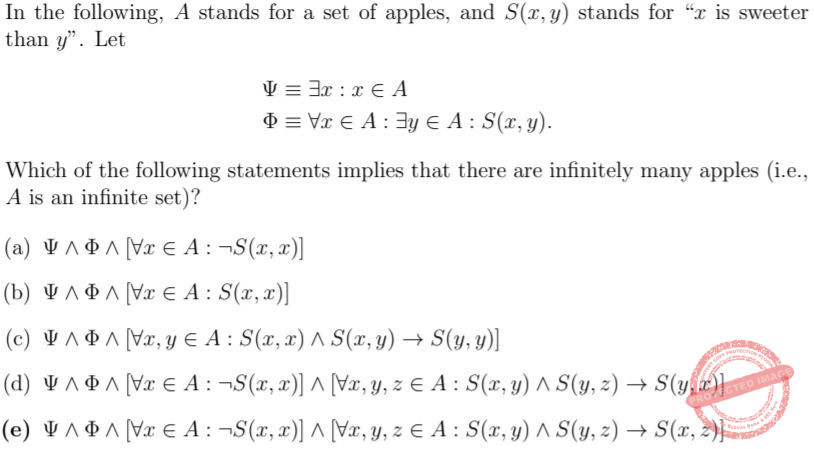A a B b C c D d E e
Engineering-Mathematics       Propositional-Logic       TIFR PHD CS & SS 2016
 Question 135
What is logically equivalent to “If Kareena and Parineeti go to the shopping mall then it is raining”:
 A If Kareena and Parineeti do not go to the shopping mall then it is not raining. B If Kareena and Parineeti do not go to the shopping mall then it is raining. C If it is raining then Kareena and Parineeti go to the shopping mall. D If it is not raining then Kareena and Parineeti do not go to the shopping mall. E None of the above
Engineering-Mathematics       Propositional-Logic       TIFR PHD CS & SS 2015
 Question 136
If Mr.M is guilty, then no witness is lying unless he is afraid. There is a witness who is afraid. Which of the following statements is true?
(Hint: Formulate the problem using the following predicates
G – Mr. M is guilty
W(x) – x is a witness
L(x) – x is lying
A(x) – x is afraid )
 A Mr.M is guilty B Mr.M is not guilty C From these facts one cannot conclude that Mr.M is guilty D There is a witness who is lying E No witness is lying.
Engineering-Mathematics       Propositional-Logic       TIFR PHD CS & SS 2012
 Question 137
For a person p, let w(p), A(p, y), L(p) and J(p) denote that p is a woman, p admires y, p is a lawyer and p is a judge respectively. Which of the following is the correct translation in first order logic of the sentence: “All women who are lawyers admire some judge”?
 A ∀x : [(w(x) ∧ L(x)) ⇒ (∃y : (J(y) ∧ w(y) ∧ A(x, y)))] B ∀x : [(w(x) ⇒ L(x)) ⇒ (∃y : (J(y) ∧ A(x, y)))] C ∀x∀y : [(w(x) ∧ L(x)) ⇒ (J(y) ∧ A(x, y))] D ∃y∀x : [(w(x) ∧ L(x)) ⇒ (J(y) ∧ A(x, y))] E ∀x : [(w(x) ∧ L(x)) ⇒ (∃y : (J(y) ∧ A(x, y)))]
Engineering-Mathematics       Propositional-Logic       TIFR PHD CS & SS 2012
 Question 138

Let n=4224165165. Consider the statements p,q,r and s as below:

P: 3 divides n

Q: 5 divides n

R: 7 divides n

S: 11 divides n

Which of the following logical expressions is TRUE?
 A P’ ⋀ q ⋀ r ⋀ s B P ⋀ q’ ⋀ r ⋀ s C P ⋀ q ⋀ r’ ⋀ s D P ⋀ q’ ⋀ r ⋀ s’
Engineering-Mathematics       Propositional-Logic       HCU PHD CS 2018 December
Question 138 Explanation:
First let’s find the truth value of P, Q, R, S.
n = 4224165165
P = 3 divides n = True
Q = 5 divides n = True
R = 7 divides n = False
S = 11 divides n = True
Now, let’s check option wise,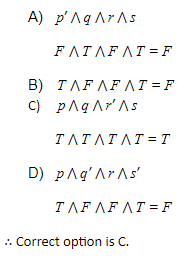Question 139
Give the negation of the following statement: For some n, for every word w in the dictionary L, w has at least n meanings.
 A For some n, there is a word w in the dictionary L, w has at least n meanings B For some n, there is at least one word w in the dictionary L, w has at most n meanings. C Given any n, all the words w in the dictionary L have at most n meanings. D Given any n, there is at least one word w in the dictionary L that has at most n meanings. E None of the given options is correct.
Engineering-Mathematics       Propositional-Logic       HCU PHD CS 2018 June
Question 139 Explanation:Question 140
p → q is logically equivalent to
 A ~p V Q B p V ~q C p V q D ~p V ~q
Engineering-Mathematics       Propositional-Logic       HCU PHD CS MAY 2016
Question 140 Explanation:
p → q is logically equivalent to ~p V Q
 Question 141
The logical expression ((A ⋀ B)' ⇒ (C' ⋀ A)) ⇒ (A ≡ 1) is:
 A A contradiction (always false) B A regular expression C A tautology (always true) D None of the above.
Engineering-Mathematics       Propositional-Logic       HCU PHD CS MAY 2013
Question 141 Explanation:
We know that if A⇒B and if B is true then whatever the A is the complete expression is always true.
Hence in question it is clearly given that the right side of ’ ⇒’ is 1(or true).Hence the complete expression is always true.
 Question 142
Consider the statement below. A person who is radical (R) is electable (E) if he/she is conservative (C), but otherwise is not electable. Few probable logical assertions of the above sentence are given below.,Which of the above logical assertions are true?
Choose the correct answer from the options given below:
 A (B) only B (C) only C (A) and (C) only D (B) and (D) only
Artificial-intelligence       Propositional-Logic       UGC NET JRF November 2020 Paper-2
Question 142 Explanation:
1. (R ∧E) ↔C
This is not equivalent. It says that all (and only) conservatives are radical and electable.
2. R →(E ↔C)
This one is equivalent. if a person is a radical then they are electable if and only if they are conservative.
3. R →((C →E) ∨¬E)
This one is vacuous. It’s equivalent to ¬R ∨ (¬C ∨ E ∨ ¬E), which is true in all interpretations.
4.R ⇒ (E ⇐⇒ C) ≡ R ⇒ ((E ⇒ C) ∧ (C ⇒ E))
≡ ¬R ∨ ((¬E ∨ C) ∧ (¬C ∨ E))
≡ (¬R ∨ ¬E ∨ C) ∧ (¬R ∨ ¬C ∨ E))
 Question 143
Consider the following argument with premiseA This is a valid argument. B Steps (C) and (E) are not correct inferences C Steps (D) and (F) are not correct inferences D Step (G) is not a correct inference
Artificial-intelligence       Propositional-Logic       UGC NET JRF November 2020 Paper-2
 Question 144
Which of the following pairs of propositions are not logically equivalent?
 ABCDArtificial-intelligence       Propositional-Logic       UGC NET JRF November 2020 Paper-2
Question 144 Explanation: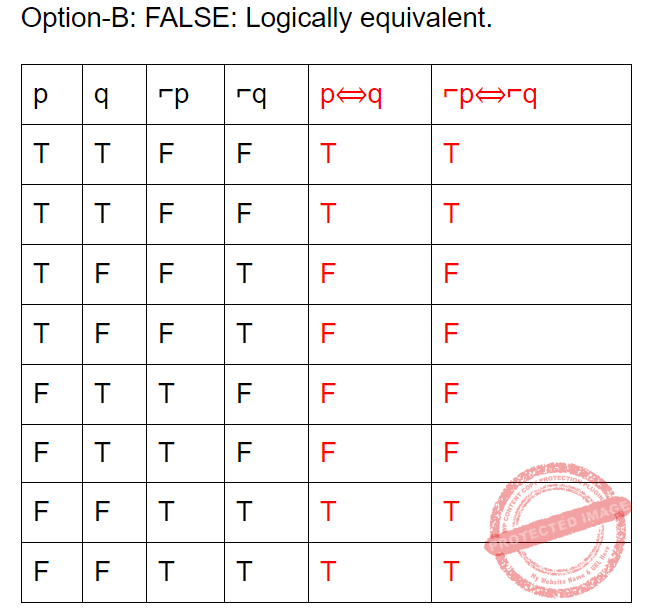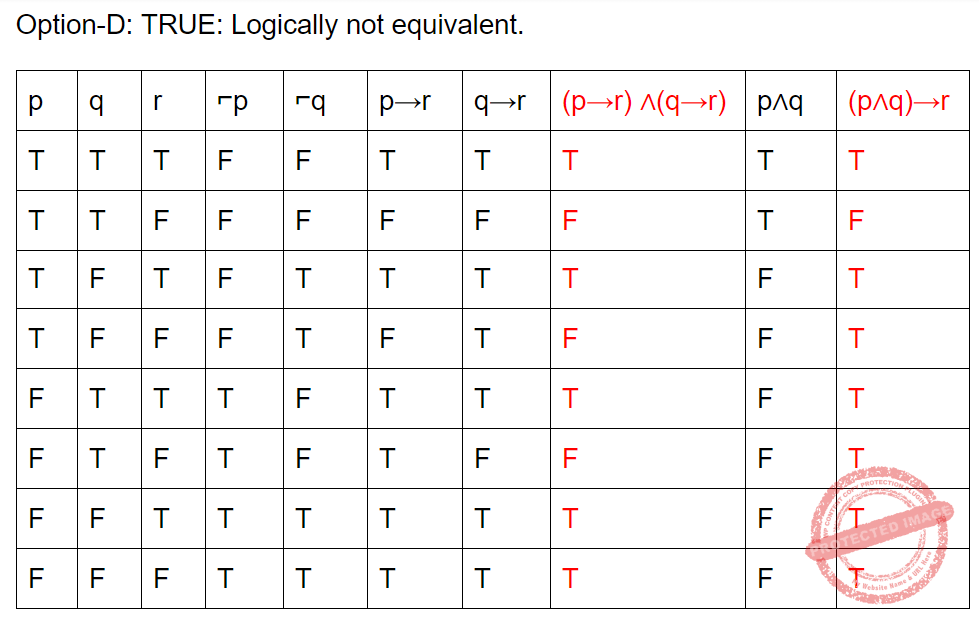Question 145
If f(x)=x is my friend, and p(x) = x is perfect, then the correct logical translation of the statement "some of my friends are not perfect" is _____.
 ABCD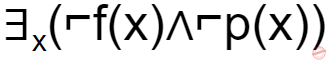Artificial-intelligence       Propositional-Logic       UGC NET JRF November 2020 Paper-2
Question 145 Explanation:
Input:
f(x)=x is my friend
p(x) = x is perfect
So, they are asking about SOME. Finally, outer most parentheses will get SOME.
So, based on this we will eliminate 2 options.
They are given conditions like NOT perfect. So, we get ⌐p(x).
The final condition is ∃x(f(x)∧⌐p(x))
 Question 146
Some children are given boxes containing sweets. Harish is happy if he gets either gems or toffees. Rekha is happy if she gets both bubble gums and peppermints. Some of the boxes are special, which means that if the box contains either gems or toffees, then it also contains bubble gums and peppermints. If Harish and Rekha are given boxes that are not special, which of the following can we infer?
 A Harish is happy B No bubble gums in Rekha’s box C No toffees in Harish’s box D There are peppermints in Rekha’s box
Engineering-Mathematics       Propositional-Logic       CMI 2020
Question 146 Explanation:
Let t denote that a box has toffees, g denote that it has gems, b denote the presence of bubble gums and p the presence of peppermints. If a box is special, it satisfies (g ∨ t) ⇒ (b ∧ p). A non-special box satisfies (g ∨ t) ∧ (¬b ∨ ¬p). Since g ∨ t holds, Harish is happy and (a) can be inferred. Since it is possible
 Question 147
If the milkman doesn’t deliver milk or the geyser doesn’t work, then Akash will be late for school and lunch will be cooked late. Suppose lunch was actually cooked on time. Which of the following is definitely true?
 A Akash was late for school B Akash reached school in time C Geyser worked D Milkman did not deliver milk
Engineering-Mathematics       Propositional-Logic       CMI 2021
Question 147 Explanation:
If either the milkman doesn’t deliver milk or the geyser doesn’t work, then (in partic- ular) lunch will be cooked late. But lunch was actually cooked on time. So it follows that the milkman delivered on time and the geyser actually worked. So option (c) is definitely true, and option (d) is definitely false. Akash can either be a dutiful student and reach school on time, or be lazy and not go to school on time. So neither (a) nor (b) can be asserted for certain.
There are 147 questions to complete.